# Overview

This document introduces the usage of CHNOSZ, a package for the R software environment. For more information on R, see “An Introduction to R” and the contributed documentation for R.

CHNOSZ has been developed since 2006 as a tool for thermodynamic calculations in geochemistry and compositional biology. The package provides functions and a thermodynamic database that can be used to calculate the stoichiometric and energetic properties of reactions involving minerals and inorganic and/or organic aqueous species. These functions also enable calculations of chemical affinities and metastable equilibrium distributions of proteins. A major feature of the package is the production of diagrams to visualize the effects of changing temperature, pressure, and activities of basis species on the potential for reactions among various species.

After starting R, install CHNOSZ by selecting the “Install packages from CRAN” or similar menu item in the R GUI or by using the following command: Or, install the package from a package file, which you can download from CRAN or (for the development version) from R-Forge.

``install.packages("CHNOSZ")``

Then load the CHNOSZ package to make its data and functions available in your R session:

``library(CHNOSZ)``
```## CHNOSZ version 1.3.2 (2019-04-20)
```
```## reset: creating "thermo" object
```
```## obigt: loading default database with 1841 aqueous, 3360 total species
```

CHNOSZ is now ready to go with the default thermodynamic database and an empty system definition. After running some calculations, you may want to “start over” with the default values. To clear the system settings and restore the default thermodynamic database, use `reset()`.

``reset()``
```## reset: resetting "thermo" object
```
```## obigt: loading default database with 1841 aqueous, 3360 total species
```

Note: Throughout this document, syntax highlighting is applied to the input of the code chunks. Double hash marks (`##`) precede the output, where black text denotes results and blue text is used for messages.

## Getting help

After CHNOSZ is installed, type `help.start()` to browse the R help documents, then choose “Packages” followed by “CHNOSZ”. That shows an index of the manual (help pages) for each function; many of the help pages include examples. There are also links to the demos (longer examples) and vignettes (more in-depth documentation; this document is a vignette).

Suggestions for accessing the documentation are indicated here with blue text. For example, read `?`CHNOSZ-package`` to get an overview of the package and a list of features. `?`” is a shortcut to R’s `help()` function. The command here is equivalent to `help(“CHNOSZ-package”)`.

## Organization of major functions

CHNOSZ is made up of a set of functions and supporting datasets. The major components of the package are shown in the figure below, which is an updated version of the diagram in Dick (2008Dick JM. 2008. Calculation of the relative metastabilities of proteins using the CHNOSZ software package. Geochemical Transactions 9: 10. doi: 10.1186/1467-4866-9-10). Rectangles and ellipses represent functions and datasets; bold text indicates primary functions.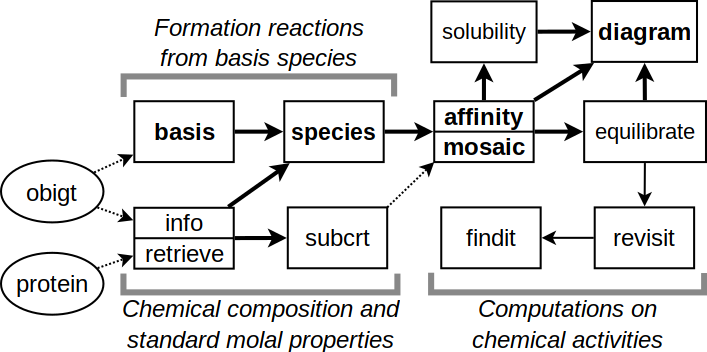Structure of CHNOSZ.

Many functions in CHNOSZ have no side effects. That is, the function only returns a result; to use the result elsewhere, it can be assigned to a variable with `<-`. In this document, the names of these functions are shown in green text (not applicable to the code chunks). When they are mentioned, names of functions in the base and recommended packages of R are said to belong to R. Example: Use R’s `plot()` to plot the data. Major functions without side effects in CHNOSZ are:

• `info()`: search for species in the thermodynamic database;
• `subcrt()`: calculate the thermodynamic properties of species and reactions;
• `affinity()`: calculate the affinities of formation reactions using given chemical activities;
• `equilibrate()`: calculate the equilibrium chemical activities of the species of interest;
• `diagram()`: plot the results.

Some functions in CHNOSZ do have side effects: they modify the `thermo` data object in the current R session. In this document, the names of these functions are shown in red text (but not in the code chunks). Major functions with side effects are:

• `basis()`: set the basis species and their chemical activities;
• `species()`: set the species of interest and their (non-equilibrium) chemical activities;
• `reset()`: reset the database, restoring all settings to their default values.

The following pseudocode shows a common sequence of commands. In actual usage, the `...` are replaced by arguments that define the chemical species and variables:

``````reset()         ## initialize system settings
basis(...)
species(...)
a <- affinity(...)
e <- equilibrate(a)  ## optional
diagram(e)           ## or diagram(a)
reset()         ## clear settings for next calculation``````

# The basics

• Use `info()` to search the thermodynamic database.
``info("aden ")``
```## info.approx: 'aden ' is ambiguous; has approximate matches to 6 species:
```
```##  "adenine"        "adenosine"      "deoxyadenosine" "adenine"
```
``##  NA``
``info("adenine")``
```## info.character: found adenine(aq); other available state is cr
```
``##  1575``
``iadenine <- info("adenine")``
```## info.character: found adenine(aq); other available state is cr
```
``info(iadenine)``
``````##         name abbrv formula state        ref1  ref2      date     G     H     S
## 1575 adenine  <NA>  C5H5N5    aq LH06a [S07] LCT17 26.Jul.17 74770 31235 53.41
##         Cp    V    a1 a2     a3 a4    c1     c2   omega Z
## 1575 51.63 90.6 2.353  0 -17.75  0 48.54 -33180 -109300 0``````
• Use `thermo.refs()` to look up references.
``thermo.refs(iadenine)``
``````##       key                                   author year
## 134 LH06a          D. E. LaRowe and H. C. Helgeson 2006
## 199 LCT17 A. R. Lowe, J. S. Cox and P. R. Tremaine 2017
##                                   citation
## 134 Geochim. Cosmochim. Acta 70, 4680-4724
## 199   J. Chem. Thermodynamics 112, 129-145
##                                                 note
## 134 nucleic-acid bases, nucleosides, and nucleotides
##                                           URL
## 134 https://doi.org/10.1016/j.gca.2006.04.010
## 199 https://doi.org/10.1016/j.jct.2017.04.005``````
• Use `subcrt()` to calculate standard molal thermodynamic properties.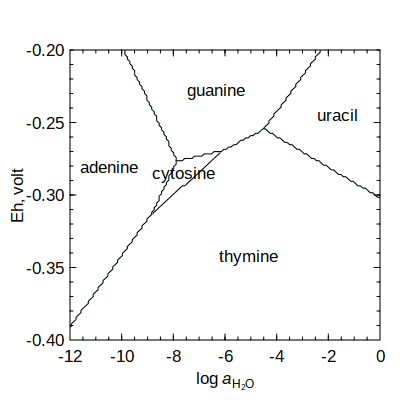Nucleobase equal-activity diagram at T = 100 °C.

``subcrt("adenine", T = 100)``
```## info.character: found adenine(aq); other available state is cr
```
```## subcrt: 1 species at 373.15 K and 1.01 bar (wet)
```
``````## \$species
##         name formula state ispecies
## 1575 adenine  C5H5N5    aq     1575
##
## \$out
##     T       P      rho     logK     G       H       S       V      Cp
## 1 100 1.01322 0.958393 -41.1609 70279 35542.8 66.2548 98.5326 62.4799``````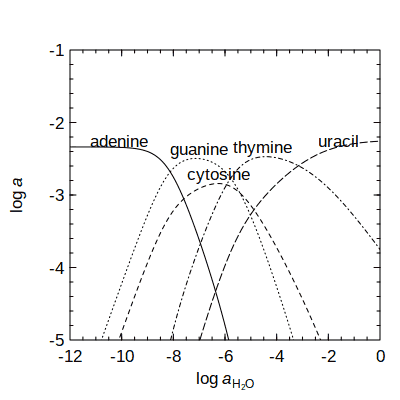Activities of nucleobases in metastable equilibrium at T = 100 °C.

• Use `basis()``species()``affinity()``diagram()` (BSAD) to construct equal-activity (equipotential) diagrams.
``````basis("CHNOSe")
a <- affinity(H2O = c(-12, -0), Eh = c(-0.4, -0.2), T = 100)
diagram(a)``````
• Use `equilibrate()` to calculate equilibrium activities.
``````basis("e-", 3.6)
a <- affinity(H2O = c(-12, 0), T = 100)
e <- equilibrate(a)
diagram(e, ylim = c(-5, -1))``````

# Thermodynamic database

An attempt has been made to provide a primary database (OBIGT) that has no major inconsistencies. As the database includes datasets from many sources, it can not be guaranteed to be fully internally consistent. For crucial problems, check not only the accuracy of entries in the database, but also the suitability of the data for your problem. If there are any doubts, consult the primary sources. Use `thermo.refs()` to show a list of references for the data; see also the vignette, Thermodynamic data in CHNOSZ, for more information.

## The `info()` function

`info()` provides an interface to the thermodynamic database packaged with CHNOSZ. Suppose you are interested in the thermodynamic properties of aqueous methane. You can search for the species by name:

``info("methane")``
```## info.character: found methane(aq); other available states are liq, gas
```
``##  840``

The number that is returned can be used to identify the species for other functions in CHNOSZ. Multiple entries exist for methane; the index of the `aq` (aqueous) species is returned by default. This convention applies to organic species, but for inorganic species, the English name refers to the gas (`info(“oxygen”)`) while the chemical formula is used to identify the aqueous species (`info(“O2”)`). A second argument can be used to specify a different physical state:

``info("methane", "gas")``
``##  3074``

Taking the species number of aqueous methane returned by `info()`, use the function again to retrieve the set of standard molal thermodynamic properties and equations of state parameters:

``````imethane <- info("methane")
info(imethane)``````
``````##        name abbrv formula state       ref1 ref2      date     G      H  S    Cp
## 840 methane  <NA>     CH4    aq PS01 [S07] <NA> 04.Oct.00 -8140 -20930 21 60.23
##      V    a1    a2     a3     a4    c1    c2  omega Z
## 840 36 1.769 -1530 -67.88 114700 40.87 64500 -40000 0``````

Liquid water is species number 1; it has NA entries in the database because specialized functions are used to compute its properties:

``info(info("water"))``
``````##    name abbrv formula state ref1 ref2      date  G  H  S Cp  V  a  b  c  d  e
## 1 water  <NA>     H2O   liq <NA> <NA> 25.Oct.06 NA NA NA NA NA NA NA NA NA NA
##    f lambda  T
## 1 NA     NA NA``````

## Fuzzy searches

Calling `info()` with a string that does not exactly match the name of any species invokes a fuzzy search of the database:

``info("acid")``
```## info.approx: 'acid' is ambiguous; has approximate matches to 83 species:
```
```##   "a-aminobutyric acid"     "acetamide"               "formic acid"             "acetic acid"             "propanoic acid"          "n-butanoic acid"
##   "n-pentanoic acid"        "n-hexanoic acid"         "n-heptanoic acid"        "n-octanoic acid"         "n-nonanoic acid"         "n-decanoic acid"
##  "n-undecanoic acid"       "n-dodecanoic acid"       "n-benzoic acid"          "o-toluic acid"           "m-toluic acid"           "p-toluic acid"
##  "oxalic acid"             "malonic acid"            "succinic acid"           "glutaric acid"           "adipic acid"             "pimelic acid"
##  "suberic acid"            "azelaic acid"            "sebacic acid"            "glycolic acid"           "lactic acid"             "2-hydroxybutanoic acid"
##  "2-hydroxypentanoic acid" "2-hydroxyhexanoic acid"  "2-hydroxyheptanoic acid" "2-hydroxyoctanoic acid"  "2-hydroxynonanoic acid"  "2-hydroxydecanoic acid"
##  "aspartic acid"           "glutamic acid"           "cis-aconitic acid"       "isocitric acid"          "a-ketoglutaric acid"     "fumaric acid"
##  "malic acid"              "oxaloacetic acid"        "pyruvic acid"            "uracil"                  "citric acid"             "metacinnabar"
##  "acide fulvique"          "acide humique"           "aspartic acid"           "glutamic acid"           "2-iodobenzoic acid"      "3-iodobenzoic acid"
##  "4-iodobenzoic acid"      "uracil"                  "phosphoric acid"         "citric acid"             "acetamide"               "nicotinamide,red"
##  "nicotinamide,ox"         "acetic acid"             "propanoic acid"          "n-butanoic acid"         "n-pentanoic acid"        "n-hexanoic acid"
##  "n-heptanoic acid"        "n-octanoic acid"         "n-nonanoic acid"         "n-decanoic acid"         "n-undecanoic acid"       "n-dodecanoic acid"
##  "n-nonadecanoic acid"     "n-eicosanoic acid"       "2-iodobenzoic acid"      "3-iodobenzoic acid"      "4-iodobenzoic acid"
```
``##  NA``

The message includes e.g. “uracil” and “metacinnabar” because their names have some similarity to the search term. Since “ribose” is the name of a species in the database, to find species with similar names, add an extra character to the search:

``info(" ribose")``
```## info.approx: ' ribose' is ambiguous; has approximate matches to 8 species:
```
```##  "ribose"               "deoxyribose"          "ribose-5-phosphate"
##  "ribose-5-phosphate-1" "ribose-5-phosphate-2" "ribose"
##  "deoxyribose"          "ribose-5-phosphate"
```
``##  NA``

The messages may be useful for browsing the database, but owing to their ambiguous results, these fuzzy searches return an `NA` value for the species index.

## Counting elements, chemical formulas, `ZC()`

Continuing with the example of methane, let’s look at its chemical formula:

``info(imethane)\$formula``
``##  "CH4"``

We can use `makeup()` to count the elements in the formula, followed by `as.chemical.formula()` to rewrite the formula on one line:

``makeup(imethane)``
``````## C H
## 1 4``````
``as.chemical.formula(makeup(imethane))``
``##  "CH4"``

For organic species, a calculation of the average oxidation state of carbon (ZC) is possible given the species index, chemical formula, or elemental count:

``ZC(imethane)``
``##  -4``
``ZC(info(imethane)\$formula)``
``##  -4``
``ZC(makeup(imethane))``
``##  -4``

# Calculating thermodynamic properties

To calculate the standard molal properties of species and reactions, use `subcrt()`. The inspiration for the name `subcrt()`, and the source of the Fortran subroutine used to calculate the thermodynamic properties of H2O, is SUPCRT (Johnson et al., 1992). (1992Johnson JW, Oelkers EH, Helgeson HC. 1992. SUPCRT92: A software package for calculating the standard molal thermodynamic properties of minerals, gases, aqueous species, and reactions from 1 to 5000 bar and 0 to 1000°C. Computers & Geosciences 18(7): 899–947. doi: 10.1016/0098-3004(92)90029-Q) If no reaction coefficients are given, `subcrt()` calculates the standard molal properties of individual species:

``subcrt("water")``
```## subcrt: 1 species at 15 values of T and P (wet)
```
``````## \$species
##    name formula state ispecies
## 1 water     H2O   liq        1
##
## \$out
## \$out\$water
##         T         P      rho    logK        G        H       S       V      Cp
## 1    0.01   1.00000 0.999829 45.0353 -56289.5 -68767.7 15.1324 18.0183 18.2056
## 2   25.00   1.00000 0.997061 41.5525 -56687.7 -68316.8 16.7123 18.0683 18.0116
## 3   50.00   1.00000 0.988030 38.6328 -57123.9 -67866.5 18.1623 18.2335 18.0046
## 4   75.00   1.00000 0.974864 36.1544 -57594.9 -67416.1 19.5048 18.4797 18.0416
## 5  100.00   1.01322 0.958393 34.0270 -58098.4 -66963.8 20.7596 18.7973 18.1579
## 6  125.00   2.32014 0.939073 32.1832 -58631.7 -66507.3 21.9419 19.1840 18.3333
## 7  150.00   4.75717 0.917058 30.5718 -59193.3 -66045.6 23.0640 19.6446 18.5664
## 8  175.00   8.91805 0.892343 29.1531 -59781.4 -65576.6 24.1360 20.1887 18.8830
## 9  200.00  15.53650 0.864743 27.8960 -60394.5 -65098.0 25.1682 20.8330 19.3288
## 10 225.00  25.47860 0.833873 26.7753 -61031.2 -64605.9 26.1712 21.6042 19.9704
## 11 250.00  39.73649 0.799072 25.7711 -61690.3 -64095.0 27.1569 22.5452 20.9123
## 12 275.00  59.43125 0.759236 24.8670 -62370.7 -63557.5 28.1400 23.7281 22.3513
## 13 300.00  85.83784 0.712408 24.0494 -63071.1 -62980.9 29.1407 25.2878 24.7394
## 14 325.00 120.45757 0.654577 23.3072 -63790.8 -62341.4 30.1952 27.5219 29.4475
## 15 350.00 165.21129 0.574688 22.6310 -64528.9 -61575.6 31.3971 31.3478 43.5985``````

That uses the default temperature and pressure settings, i.e. equally spaced temperature intervals from 0 to 350 °C at Psat. Psat is 1 bar below 100 °C, or the pressure of liquid-vapor saturation (i.e. boiling) at higher temperatures. The columns in the output are temperature, pressure, density of water, logarithm of the equilibrium constant (only meaningful for reactions; see below), standard molal Gibbs energy and enthalpy of formation from the elements, standard molal entropy, volume, and heat capacity. The corresponding units are °C (`T`), bar (`P`), g cm-3 (`rho`), cal mol-1 (`G` and `H`), cal K-1 mol-1 (`S` and `Cp`), and cm3 mol-1 (`V`).

A custom temperature-pressure grid can be specified. Here, we calculate the properties of H2O on a T, P grid in the supercritical region, with conditions grouped by pressure: See also `demo(density)`.

``subcrt("water", T = c(400, 500, 600), P = c(200, 400, 600), grid = "P")\$out\$water``
```## subcrt: 1 species at 9 values of T and P (wet)
```
``````##     T   P       rho    logK        G        H       S        V      Cp
## 1 400 200 0.1005447 21.5271 -66306.4 -56639.3 39.0387 179.1760 27.4305
## 2 500 200 0.0677109 19.8868 -70353.7 -54819.8 41.5776 266.0607 14.0774
## 3 600 200 0.0550386 18.6704 -74593.1 -53540.0 43.1368 327.3197 11.9607
## 4 400 400 0.5236661 21.4119 -65951.4 -60454.4 32.8438  34.4021 37.5317
## 5 500 400 0.1779739 19.6639 -69565.0 -56252.5 38.7044 101.2238 24.9675
## 6 600 400 0.1238075 18.4124 -73562.4 -54361.7 41.0152 145.5098 15.4901
## 7 400 600 0.6124511 21.3631 -65801.3 -60832.4 32.0592  29.4149 25.8810
## 8 500 600 0.3384430 19.5660 -69218.6 -57694.2 36.3916  53.2296 32.4385
## 9 600 600 0.2071976 18.2784 -73027.2 -55195.1 39.4479  86.9469 19.3763``````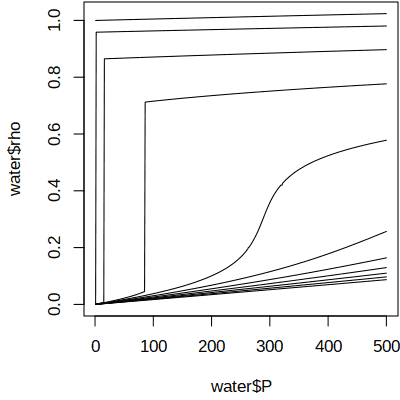Isothermal contours of density (g cm-3) and pressure (bar) of water.

The additional operations (`\$out\$water`) are used to extract a specific part of the results; this can be used with e.g. R’s `write.table()` or `plot()` for further processing:

``````substuff <- subcrt("water", T=seq(0,1000,100), P=c(NA, seq(1,500,1)), grid="T")
water <- substuff\$out\$water
plot(water\$P, water\$rho, type = "l")``````

## Changing units

The default units of temperature, pressure, and energy are °C, bar, and calories. The functions `T.units()`, `P.units()`, and `E.units()` can be used to change the units used by various functions in CHNOSZ. What is the Gibbs energy in J/mol of aqueous methane at 298.15 K and 0.1 MPa?

``````T.units("K")
P.units("MPa")
E.units("J")
subcrt("methane", T = 298.15, P = 0.1)\$out\$methane\$G``````
``##  -34057.8``

A related function, `convert()`, can be used to convert given values between units. Let’s convert the standard Gibbs energy of aqueous methane listed in the database from cal/mol to J/mol:

``convert(info(info("methane"))\$G, "J")``
``##  -34057.8``

As expected, we get the same result from both operations.

Use `reset()` to restore the units and all other settings for CHNOSZ to their defaults:

``reset()``
```## reset: resetting "thermo" object
```
```## obigt: loading default database with 1841 aqueous, 3360 total species
```

# Properties of reactions

## Reaction definitions

To calculate the thermodynamic properties of reactions, give the names of species, the physical states (optional), and reaction coefficients as the arguments to `subcrt()`. Here we calculate properties for the dissolution of CO2: Because of aqueous speciation, this doesn’t give the solubility of CO2. Some examples of solubility calculations are in `demo(solubility)` (see below).

``subcrt(c("CO2", "CO2"), c("gas", "aq"), c(-1, 1), T = seq(0, 250, 50))``
```## subcrt: 2 species at 6 values of T and P (wet)
```
``````## \$reaction
##      coeff           name formula state ispecies
## 3075    -1 carbon dioxide     CO2   gas     3075
## 1479     1            CO2     CO2    aq     1479
##
## \$out
##        T        P      rho     logK       G         H         S       V      Cp
## 1   0.01  1.00000 0.999829 -1.10873 1385.80 -5907.949 -26.70130 23.6978 49.1458
## 2  50.00  1.00000 0.988030 -1.71865 2541.27 -3947.724 -20.08036 36.9843 34.6260
## 3 100.00  1.01322 0.958393 -2.00354 3420.89 -2271.995 -15.25623 41.5584 33.0446
## 4 150.00  4.75717 0.917058 -2.10770 4080.94  -596.111 -11.05290 44.5642 34.3421
## 5 200.00 15.53650 0.864743 -2.09920 4544.74  1228.815  -7.00814 47.9553 39.3857
## 6 250.00 39.73649 0.799072 -2.01184 4815.90  3513.101  -2.49026 54.3882 55.7180``````

In order to make a plot like Figure 18 of Manning et al. (2013Manning CE, Shock EL, Sverjensky DA. 2013. The chemistry of carbon in aqueous fluids at crustal and upper-mantle conditions: Experimental and theoretical constraints. Reviews in Mineralogy and Geochemistry 75(1): 109–148. doi: 10.2138/rmg.2013.75.5), let’s run more calculations and store the results. In addition to the reaction definition, we specify a greater number of temperature points than the default: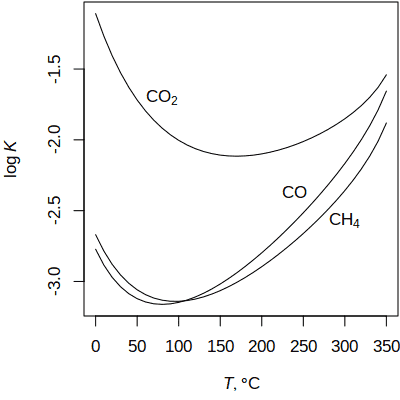Calculated equilibrium constants for dissolution of CO2, CO, and CH4.

``````T <- seq(0, 350, 10)
CO2 <- subcrt(c("CO2", "CO2"), c("gas", "aq"), c(-1, 1), T = T)\$out\$logK
CO <- subcrt(c("CO", "CO"), c("gas", "aq"), c(-1, 1), T = T)\$out\$logK
CH4 <- subcrt(c("CH4", "CH4"), c("gas", "aq"), c(-1, 1), T = T)\$out\$logK
logK <- data.frame(T, CO2, CO, CH4)``````

Now we can make the plot, using R’s `matplot()`. Here, `axis.label()` and `expr.species()` are used to create formatted axis labels and chemical formulas:

``````matplot(logK[, 1], logK[, -1], type = "l", col = 1, lty = 1,
xlab = axis.label("T"), ylab = axis.label("logK"))
text(80, -1.7, expr.species("CO2"))
text(240, -2.37, expr.species("CO"))
text(300, -2.57, expr.species("CH4"))``````

## Unbalanced reactions

A balanced chemical reaction conserves mass. `subcrt()` won’t stop you from running an unbalanced reaction, but it will give you a warning:

``subcrt(c("CO2", "CH4"), c(-1, 1))``
```## info.character: found CO2(aq); other available state is gas
```
```## info.character: found CH4(aq); other available states are liq, gas
```
```## subcrt: 2 species at 15 values of T and P (wet)
```
```## subcrt: reaction is not balanced; it is missing this composition:
```
```##  H  O
## -4  2
```
```## Warning in subcrt(c("CO2", "CH4"), c(-1, 1)): reaction was unbalanced, missing
## H-4O2
```

In other words, to balance the reaction, we should add 4 H to the left and 2 O to the right. That could be done manually be redefining the reaction with the appropriate species. There is another option: balancing the reaction automatically using basis species.

## Setting the basis species

Basis species are a minimal number of chemical species that linearly combine to give the composition of any species in the system. The basis species are similar to thermodynamic components, but can include charged species. Basis species are used in CHNOSZ to automatically balance reactions; they are also required for making chemical activity diagrams.

``basis(c("CO2", "H2", "H2CO2"))``
```## Error in put.basis(ispecies, logact): singular stoichiometric matrix
```

That set of species has a singular (non-invertible) stoichiometric matrix. An error would also result from either an underdetermined or overdetermined system. A valid set of basis species has an invertible stoichiometric matrix and the same number of species as elements:

``basis(c("CO2", "H2", "H2O"))``
``````##     C H O ispecies logact state
## CO2 1 0 2     1479      0    aq
## H2  0 2 0       62      0    aq
## H2O 0 2 1        1      0   liq``````

The composition of any species made up of C, H, and O can be represented by a single linear combination of these basis species.

## Automatically balancing reactions

Methanogenic metabolism in reducing environments may proceed by acetoclastic or hydrogenotrophic processes. To consider reactions involving a charged species (acetate), let’s define a basis with H+:

``basis(c("CO2", "H2", "H2O", "H+"))``
``````##     C H O Z ispecies logact state
## CO2 1 0 2 0     1479      0    aq
## H2  0 2 0 0       62      0    aq
## H2O 0 2 1 0        1      0   liq
## H+  0 1 0 1        3      0    aq``````

By identifying species other than the basis species, the reactions will be automatically balanced. This produces the balanced reaction for acetoclastic methanogenesis:

``subcrt(c("acetate", "methane"), c(-1, 1))\$reaction``
``````##      coeff    name formula state ispecies
## 1038    -1 acetate C2H3O2-    aq     1038
## 840      1 methane     CH4    aq      840
## 1479     1     CO2     CO2    aq     1479
## 3       -1      H+      H+    aq        3``````

We can similarly consider reactions for hydrogenotrophic methanogenesis as well as acetate oxidation (without production of methane):

``````acetate_oxidation <- subcrt("acetate", -1)
hydrogenotrophic <- subcrt("methane", 1)
acetoclastic <- subcrt(c("acetate", "methane"), c(-1, 1))``````

Use `describe.reaction()` to write the reactions on a plot: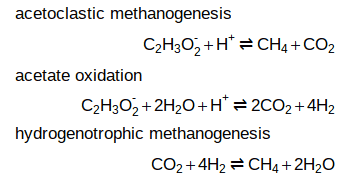``````plot(0, 0, type = "n", axes = FALSE, ann=FALSE, xlim=c(0, 5), ylim=c(5.2, -0.2))
text(0, 0, "acetoclastic methanogenesis", adj = 0)
text(5, 1, describe.reaction(acetoclastic\$reaction), adj = 1)
text(0, 2, "acetate oxidation", adj = 0)
text(5, 3, describe.reaction(acetate_oxidation\$reaction), adj = 1)
text(0, 4, "hydrogenotrophic methanogenesis", adj = 0)
text(5, 5, describe.reaction(hydrogenotrophic\$reaction), adj = 1)``````

## Chemical affinity

Usually, `subcrt()` returns only standard state thermodynamic properties. The standard state adopted for H2O is unit activity of the pure component at any T and P. The standard state for aqueous species is unit activity of a hypothetical one molal solution referenced to infinite dilution at any T and P. Thermodynamic models often consider a non-standard state (i.e. non-unit activity). The activities of basis species can be modified with `basis()`, and those of the other species using the `logact` argument in `subcrt()`.

Let us calculate the chemical affinity of acetoclastic methanogenesis. The affinity is equal to the negative of the overall (non-standard) Gibbs energy change of the reaction. We begin by changing the energy units to Joules. Then, we change the state of H2O and CO2 in the basis from `aq` (aqueous) to `gas`, and set the logarithm of fugacity of gaseous H2 and the pH, using values from Mayumi et al. (2013Mayumi D, Dolfing J, Sakata S, Maeda H, Miyagawa Y, Ikarashi M, Tamaki H, Takeuchi M, Nakatsu CH, Kamagata Y. 2013. Carbon dioxide concentration dictates alternative methanogenic pathways in oil reservoirs. Nature Communications 4: 1998. doi: 10.1038/ncomms2998). The activity of acetate and fugacity of methane, as well as temperature and pressure, are set in the call to `subcrt()`:

``````E.units("J")
basis(c("CO2", "H2", "H2O", "H+"))
basis(c("CO2", "H2"), "gas")
basis(c("H2", "pH"), c(-3.92, 7.3))``````
``````subcrt(c("acetate", "methane"), c(-1, 1),
c("aq", "gas"), logact = c(-3.4, -0.18), T = 55, P = 50)\$out``````
``````##    T  P      rho    logK        G       H       S        V      Cp  logQ
## 1 55 50 0.987821 13.5984 -85429.6 18706.9 317.685 -40.1339 39.2144 10.52
##         A
## 1 19339.4``````

The new `A` column shows the affinity; the other columns are unaffected and still show the standard-state properties. Let’s repeat the calculation for hydrogenotrophic methanogenesis.

``subcrt("methane", 1, "gas", logact = -0.18, T = 55, P = 50)\$out``
``````##    T  P      rho   logK       G       H        S       V      Cp logQ       A
## 1 55 50 0.987821 18.828 -118284 -251807 -407.195 36.4746 33.4702 15.5 20907.8``````

Under the specified conditions, the affinities of hydrogenotrophic and acetoclastic methanogenesis are somewhat greater than and less than 20 kJ, respectively. This result matches Figure 4b in Mayumi et al. (2013) at unit fugacity of CO2.

We can go even further and reproduce their plot. The reproduction is not identical, owing to differences of thermodynamic data and of calculations of the effects of temperature and pressure. To make the code neater, we write a function that can run any of the reactions:

``````rxnfun <- function(coeffs) {
subcrt(c("acetate", "methane"), coeffs,
c("aq", "gas"), logact = c(-3.4, -0.18), T = 55, P = 50)\$out
}``````

Now we’re ready to calculate and plot the affinities. Here, we use R’s `lapply()` to list the results at two values of logarithm of fugacity of CO2. We insert an empty reaction to get a line at zero affinity. R’s `do.call()` and `rbind()` are used to turn the list into a data frame that can be plotted with R’s `matplot()`. There, we plot the negative affinities, equal to Gibbs energy, as shown in the plot of Mayumi et al. (2013).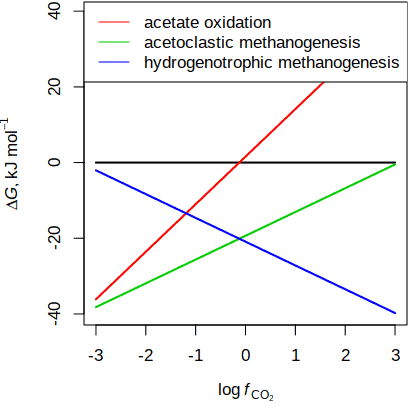Gibbs energies of acetate oxidation and methanogenesis (after Mayumi et al., 2013).

``````Adat <- lapply(c(-3, 3), function(logfCO2) {
basis("CO2", logfCO2)
data.frame(logfCO2,
rxnfun(c(0, 0))\$A,
rxnfun(c(-1, 0))\$A,
rxnfun(c(-1, 1))\$A,
rxnfun(c(0, 1))\$A
)
})
matplot(Adat[, 1], -Adat[, -1]/1000, type = "l", lty = 1, lwd = 2,
xlab = axis.label("CO2"), ylab = axis.label("DG", prefix = "k"))
legend("topleft", c("acetate oxidation", "acetoclastic methanogenesis",
"hydrogenotrophic methanogenesis"), lty = 1, col = 2:4)``````

Let’s not forget to clear the system settings, which were modified by `basis()` and `E.units()`, before running other calculations:

``reset()``

# Using `affinity()`

`affinity()` offers calculations of chemical affinity of formation reactions over a configurable range of T, P, and activities of basis species.

## Species of interest

By formation reaction is meant the stoichiometric requirements for formation of one mole of any species from the basis species. The `species()` function is used to set these species of interest. Let’s consider the stoichiometry of some aqueous sulfur-bearing species. Here we use `basis()` with a keyword to load a preset basis definition. Some available keywords are `CHNOS` (including CO2, H2O, NH3, H2S, and O2), `CHNOS+` (also including H+), and `CHNOSe` (including H+, and e- instead of O2). See `?basis` for more options. What is `SO42-`? Is it 1 S, 4 O, and 2 negative charges, or 1 S, 42 O, and 1 negative charge? The ambiguity of a digit that could belong to the coefficient for the following charge or to that for the preceding element is why formulas in CHNOSZ are written with the number of charges after the + or - symbol. `SO4-2` is unambiguously parsed as 1 S, 4 O and 2 negative charges.

``basis("CHNOS+")``
``species(c("H2S", "HS-", "HSO4-", "SO4-2"))``
``````##   CO2 H2O NH3 H2S O2 H+ ispecies logact state  name
## 1   0   0   0   1  0  0       65     -3    aq   H2S
## 2   0   0   0   1  0 -1       22     -3    aq   HS-
## 3   0   0   0   1  2 -1       25     -3    aq HSO4-
## 4   0   0   0   1  2 -2       24     -3    aq SO4-2``````

Aqueous species are assigned default activities of 10-3 (`logact` is -3). Now, we can use `affinity()` to calculate the affinities of the formation reactions of each of the species. R’s `unlist()` is used here to turn the list of values of affinity into a numeric object that can be printed in a couple of lines (note that the names correspond to `ispecies` above): The values returned by `affinity()` are dimensionless, i.e. A/(2.303RT).

``unlist(affinity()\$values)``
```## affinity: temperature is 25 C
```
```## affinity: pressure is Psat
```
```## subcrt: 10 species at 298.15 K and 1 bar (wet)
```
``````##        65        22        25        24
##  -4.00000  -3.98775 -29.48836 -24.46748``````

The same result (but expressed in units of cal/mol or J/mol) could be obtained using `subcrt()`; however, `affinity()` has the advantage of being able to perform calculations on a grid of T, P, or activities of basis species. Let’s choose a set of variables commonly used in aqueous speciation diagrams: Eh and pH. To use Eh as a variable, the electron (e-) should be in the basis. To put the electron in there, we can use a different keyword (`basis("CHNOSe")`), or swap oxygen out of the existing basis:

``swap.basis("O2", "e-")``
```## subcrt: 6 species at 298.15 K and 1 bar (wet)
## subcrt: 6 species at 298.15 K and 1 bar (wet)
```
``````##     C H N O S  Z ispecies   logact state
## CO2 1 0 0 2 0  0     1479 -3.00000    aq
## H2O 0 2 0 1 0  0        1  0.00000   liq
## NH3 0 3 1 0 0  0       64 -4.00000    aq
## H2S 0 2 0 0 1  0       65 -7.00000    aq
## e-  0 0 0 0 0 -1        2  6.22377    aq
## H+  0 1 0 0 0  1        3 -7.00000    aq``````

`swap.basis()` changed the basis species and recalculated their activities, but preserved the species of interest. That is, running `affinity()``\$values` again would give the same result.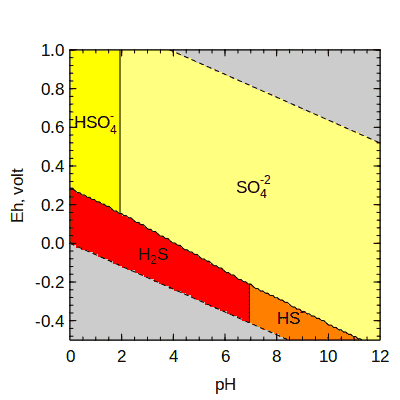Aqueous sulfur species at 25 °C.

Now we can calculate the affinities on an Eh-pH grid:

``a <- affinity(pH = c(0, 12), Eh = c(-0.5, 1))``

## Potential diagrams

Given values of affinity, the `diagram()` function uses the maximum affinity method to make a potential diagram (i.e. a Pourbaix diagram). Areas corresponding to Eh-pH conditions beyond the stability limits of water are colored slate gray. Another function, `water.lines()`, is used to draw lines at the water stability limits:

``````diagram(a, fill = "heat")
water.lines(a)``````

Note that the calculation of affinity implies a non-equilibrium reference state of equal activities of species (see above). Generally, then, `diagram()` gives a potential diagram because it shows regions of maximum affinity. In systems where equilibrium is attainable, it makes sense to call this a predominance diagram, showing regions of maximum activity.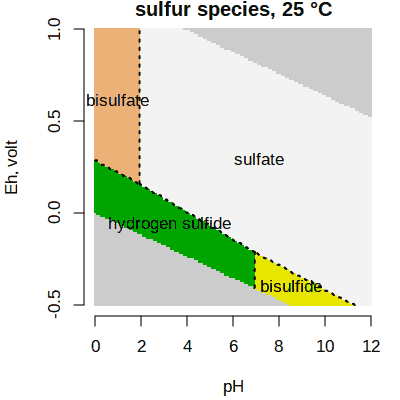The same plot, with different colors and labels.

The names of species that can be parsed as chemical formulas are formatted with subscripts and superscripts; if this is not desired, set `format.names = FALSE`. The default colors for diagrams shown on the screen use R’s `heat.colors()` palette. Some arguments in `diagram()` can be used to control the color, labels, and lines, and title. The `tplot` argument turns off plot customizations used in CHNOSZ. Additional arguments are passed to R’s plotting functions; here, we use `bty` to remove the box around the plot.

``````diagram(a, fill = "terrain", lwd = 2, lty = 3,
names = c("hydrogen sulfide", "bisulfide", "bisulfate", "sulfate"),
tplot = FALSE, main = "sulfur species, 25 °C", bty = "n")``````

Mineral stability diagrams often depict activity ratios, e.g. log (aCa+2/aH+2), on one or both axes. The variables used for potential calculations in CHNOSZ include only a single chemical activity, e.g. log aCa+2. However, you can set pH = 0 to generate diagrams that are geometrically equivalent to those calculated using activity ratios, and use `ratlab()` to make the axes labels for the ratios. Moreover, `diagram()` has a “saturation” option that can be used to draw saturation limits for minerals that do not contain the conserved basis species. See `demo(saturation)` for an example that uses activity ratios on the axes and plots saturation limits for calcite, magnesite, dolomite, and brucite on a diagram for the H2O–CO2–CaO–MgO–SiO2 system. That demo can be used as a template to produce a wide range of diagrams similar to those in Bowers et al. (1984Bowers TS, Jackson KJ, Helgeson HC. 1984. Equilibrium Activity Diagrams for Coexisting Minerals and Aqueous Solutions at Pressures and Temperatures to 5 Kb and 600C. Heidelberg: Springer-Verlag. Available at http://www.worldcat.org/oclc/11133620. ).

## Mosaic diagrams

If sulfur is in the basis species, then we should consider that its speciation is sensitive to Eh and pH, as shown in the preceding diagram. Mosaic diagrams, which are often shown for oxide, sulfide, and carbonate minerals, account for speciation of the basis species. These diagrams are made by constructing individual diagrams for the possible basis species. The individual diagrams are then combined, each one contributing to the final diagram only in the range of stability of the corresponding basis species.

Let’s use `mosaic()` to make a diagram for aqueous species and minerals in the Cu-S-Cl-H2O system. To know what aqueous copper chloride complexes are available in the database, we can use a fuzzy search:

``info(" CuCl")``
```## info.approx: ' CuCl' is ambiguous; has approximate matches to 7 species:
```
```##  "CuCl3-2" "CuCl+"   "CuCl2"   "CuCl3-"  "CuCl4-2" "CuCl"    "CuCl2-"
```

Next we define the basis, and set the activities of the H2S and Cl- basis species. These represent the total activity of S and Cl in the system, which are distributed among the minerals and aqueous species. Four minerals and the aqueous copper chloride species are included:

``````basis(c("Cu", "H2S", "Cl-", "H2O", "H+", "e-"))
basis("H2S", -6)
basis("Cl-", -0.7)
species(c("CuCl", "CuCl2-", "CuCl3-2", "CuCl+", "CuCl2", "CuCl3-", "CuCl4-2"))
species(c("chalcocite", "tenorite", "cuprite", "copper"))``````

Note that chalcocite (Cu2S) undergoes phase transitions. To get the temperatures of the phase transitions from `thermo()\$obigt` (in Kelvin, regardless of the `T.units()`), we can use `info()`. We see that at 200 °C (473.15 K) the second phase is stable; this one is automatially used by CHNOSZ for this diagram.

``info(info("chalcocite", c("cr", "cr2", "cr3")))\$T``
``##   376  717 1400``

We use `mosaic()` to generate and combine diagrams for each candidate basis species (H2S, HS-, HSO4-, or SO4-2) as a function of Eh and pH. The key argument is `bases`, which identifies the candidate basis species, starting with the one in the current basis. The other arguments, like those of `affinity()`, specify the ranges of the variables; `res` indicates the grid resolution to use for each variable (the default is 128). The first call to `diagram()` plots the species of interest; the second adds the predominance fields of the basis species. We turn off the gray coloring beyond the water stability limits (`limit.water`) but plot dashed blue lines using `water.lines()`: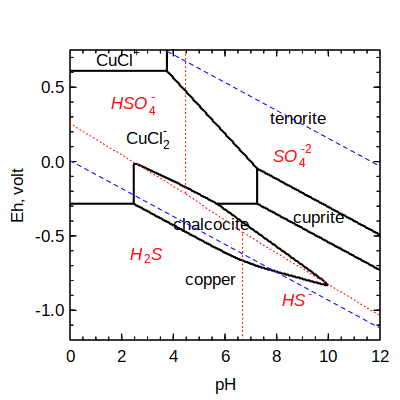Copper minerals and aqueous complexes with chloride, 200 °C.

``````T <- 200
res <- 300
bases <- c("H2S", "HS-", "HSO4-", "SO4-2")
m1 <- mosaic(bases, pH = c(0, 12, res), Eh=c(-1.2, 0.75, res), T=T)
diagram(m1\$A.species, lwd = 2, fill = NA, limit.water = FALSE)
diagram(m1\$A.bases, add = TRUE, col = "red1", col.names = "red1", lty = 3,
limit.water = FALSE, italic = TRUE)
water.lines(m1\$A.species, col = "blue1")``````

The diagrams are combined according to the relative abundances of the different possible basis species listed in `bases` along with a term for the Gibbs energy of mixing (see `?mosaic`). The smooth transitions between basis species can result in curved field boundaries, in this case around the chalcocite field. If we added the argument `blend = FALSE`, the diagrams would instead be assembled using the single predominant basis species at any point on the Eh-pH grid, and all of the line segments would be straight.

The reactions used to make this diagram are balanced on Cu, so that no Cu appears in reactions between any two other species (minerals or aqueous species). If `diagram()` is run with `balance = 1`, then the reactions are written for one mole of the mineral formulas on each side of the reaction, with the possibility of Cu appearing as an additional species to conserve the elements. This may be problematic, as Cu would be be present in some reactions in Eh-pH space where it is not a stable phase. However, it is common in low-temperature aqueous geochemical calculations to “turn off” particular redox reactions that are not thought to attain equilibrium, so decoupling a species from equilibrium may be justified in some circumstances. Changing the balance to 1 results in the loss of the tenorite stability field and extension of chalcocite stability to lower pH, as shown in Figure 5a of Caporuscio et al. (2017Caporuscio FA, Palaich SEM, Cheshire MC, Jové Colón CF. 2017. Corrosion of copper and authigenic sulfide mineral growth in hydrothermal bentonite experiments. Journal of Nuclear Materials 485: 137–146. doi: 10.1016/j.jnucmat.2016.12.036).

We have seen the effects of speciation of S in the basis species. However, the choice of other basis species can also affect the diagram. For instance, we can use H2 or O2 in place of e-. To do that, let’s write a function to swap those basis species and make a diagram. We use R’s `do.call()` to construct the argument list for `mosaic()`; this way, the name of the `newvar` argument to our function indicates the chosen variable.

``````mosaicfun <- function(newvar, T = 200) {
swap.basis("e-", names(newvar))
if (names(newvar) == "O2") basis("O2", "gas")
mosaicargs <- c(list(bases), pH = list(c(-2, 12, res)), newvar, T = T)
m1 <- do.call(mosaic, mosaicargs)
diagram(m1\$A.species, lwd = 2, fill = "terrain",
limit.water = FALSE)
diagram(m1\$A.bases, add = TRUE, col = "red1", col.names = "red1", lty = 3,
limit.water = FALSE, italic = TRUE)
water.lines(m1\$A.species, col = "blue1")
swap.basis(names(newvar), "e-")
}
par(mfrow = c(1, 3))
mosaicfun(list(Eh = c(-1, 1, res)))
mosaicfun(list(H2 = c(-30, 10, res)))
mosaicfun(list(O2 = c(-70, 5, res)))``````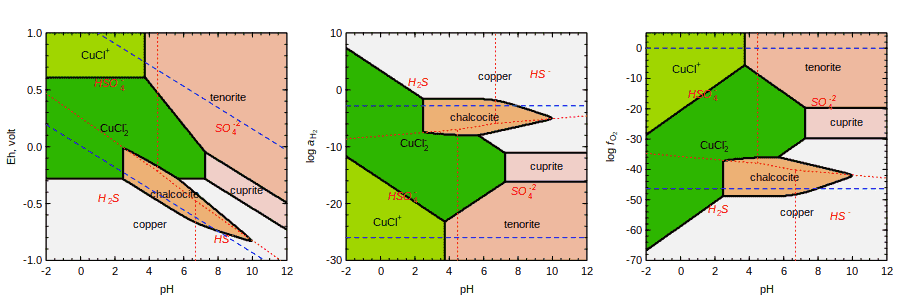The same chemical system projected into different sets of basis species.

## T, P, activity transects

Above, we used evenly-spaced grids of chemical activities of basis species; the ranges of variables were given by two or three values (minimum, maximum, and optionally resolution). `affinity()` can also perform calculations along a transect, i.e. a particular path along one or more variables. A transect is calculated when there are four or more values assigned to the variable(s). Let’s use this feature to calculate affinities (negative Gibbs energies) of methanogenesis and biosynthetic reactions in a hydrothermal system.

Some results of mixing calculations for seawater and vent fluid from the Rainbow hydrothermal field, calculated using EQ3/6 by Shock and Canovas (2010Shock E, Canovas P. 2010. The potential for abiotic organic synthesis and biosynthesis at seafloor hydrothermal systems. Geofluids 10(1-2): 161–192. doi: 10.1111/j.1468-8123.2010.00277.x), are included in a data file in CHNOSZ. Reading the file with R’s `read.csv()`, we set `check.names = FALSE` to preserve the `NH4+` column name (which is not a syntactically valid variable name):

``````file <- system.file("extdata/cpetc/SC10_Rainbow.csv", package = "CHNOSZ")
rb <- read.csv(file, check.names = FALSE)``````

We take a selection of the species from Shock and Canovas (2010) with activities equal to 10-6; methane is assigned an activity of 10-3. We will write the synthesis reactions of organic species in terms of these basis species: The constant activity of methane is a simplification of the calculation reported by Shock and Canovas (2010). The code here could be expanded to vary the activity of methane.

``````basis(c("CO2", "H2", "NH4+", "H2O", "H2S", "H+"))
species("CH4", -3)
"methane", "leucine", "tryptophan", "n-nonanoic acid"), -6)``````

Now we can calculate affinities along the transect of changing temperature and activities of five basis species. Each variable is given as a named argument; `NH4+` must be quoted. A shorter expression would use R’s `do.call()` to construct the function call: `do.call(``affinity``, as.list(rb))`. The target of the conversion is `G`, or free energy, from `logK`. That conversion requires temperature in Kelvin, which is obtained by conversion from °C. We finish with a negation (affinity is negative Gibbs energy) and scaling from cal to kcal. Using R’s `lapply()` to run `convert()` for each species, we convert the affinity from dimensionless values (A/(2.303RT)) to cal/mol, then kcal/mol.

``````a <- affinity(T = rb\$T, CO2 = rb\$CO2, H2 = rb\$H2,
`NH4+` = rb\$`NH4+`, H2S = rb\$H2S, pH = rb\$pH)
T <- convert(a\$vals[], "K")
a\$values <- lapply(a\$values, convert, "G", T)
a\$values <- lapply(a\$values, `*`, -0.001)``````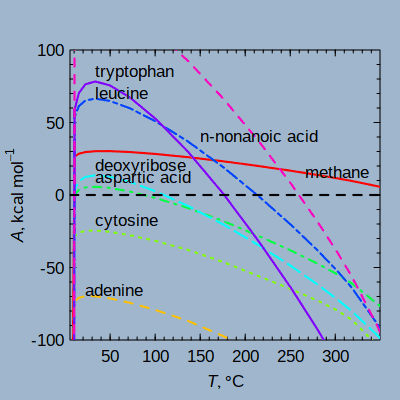Affinities of organic synthesis in a hydrothermal system, after Shock and Canovas (2010).

Finally, we use `diagram()` to plot the results. Although only temperature is shown on the x axis, pH and the activities of CO2, H2, NH4+, and H2S are also varied according to the data in `rb`. By default, `diagram()` attempts to scale the affinities by dividing by the reaction coefficients of a shared basis species (in this case, CO2). To override that behavior, we set `balance = 1` to plot the affinities of the formation reactions as written (per mole of the product species).

``````diagram(a, balance = 1, ylim = c(-100, 100), ylab = axis.label("A", prefix="k"),
col = rainbow(8), lwd = 2, bg = "slategray3")
abline(h = 0, lty = 2, lwd = 2)``````

When making line plots, `diagram()` automatically places the labels near the lines. The additional arguments `adj` and `dy` can be used to fine-tune the positions of the labels (they are used in a couple of examples below). If labeling of the lines is not desired, add e.g. `legend.x = "topright"` to make a legend instead, or `names = NULL` to prevent any plotting of the names.

## Buffers

There is one other feature of `affinity()` that can be mentioned here. Can we go the other direction: calculate the activities of basis species from the activities of the species of interest? This question relates to the concept of chemical activity buffers. In CHNOSZ there are two ways to perform buffer calculations:

1. Assign the name of a buffer (listed in `thermo()\$buffer`) to the basis species:
• more versatile (multiple activities can be buffered, e.g. both S2 and O2 by pyrite-pyrrhotite-magnetite);
• the buffers are active in calculations of affinity of other species;
• use `mod.buffer()` to change or add buffers in `thermo()\$buffer`;
• `demo(buffer)` uses it for mineral buffers (solid lines).
1. Use the `type` argument of `diagram()` to solve for the activity of the indicated basis species:
• more convenient (the buffers come from the currently defined species of interest), but only a single basis species can be buffered, and it’s not used in the calculation of affinity;
• `demo(buffer)` uses it for aqueous organic species as buffers (dotted and dashed lines).

As an example of method 1, let’s look at the pyrite-pyrrhotite-magnetite (PPM) buffer at 300 °C. For other examples, see `?buffer` and `demo(protbuff)` (hypothetical buffer made of proteins). Without the buffer, the basis species have default activities of zero. Under these conditions, the minerals are not in equilibrium, as shown by their different affinities of formation:

``````basis(c("FeS2", "H2S", "O2", "H2O"))
species(c("pyrite", "magnetite"))
species("pyrrhotite", "cr2")``````

The affinity of formation of pyrite happens to be zero because it is identical to one of the selected basis species.

``unlist(affinity(T = 300, P = 100)\$values)``
``````##     1884     2489     1886
##   0.0000 -50.6945 -20.6134``````

We use `mod.buffer()` to choose the `cr2` phase of pyrrhotite, which is stable at this temperature (see above for how to get this information for minerals with phase transitions). Then, we set up H2S and O2 to be buffered by PPM, and inspect their buffered activities:

``mod.buffer("PPM", "pyrrhotite", "cr2")``
```## mod.buffer: changed state and/or logact of pyrrhotite in PPM buffer
```
``basis(c("H2S", "O2"), c("PPM", "PPM"))``
``unlist(affinity(T = 300, P = 100, return.buffer = TRUE)[1:3])``
``````##       H2S        O2      FeS2
##  -2.36691 -36.49303   0.00000``````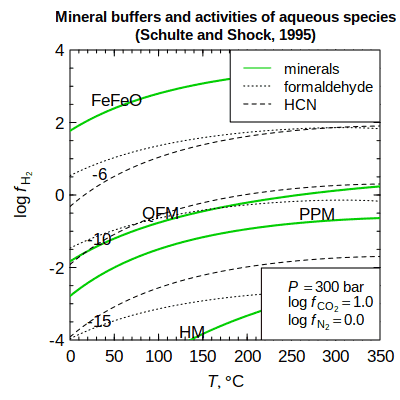Values of logfH2 corresponding to mineral buffers or to given activities of aqueous species.

Et voilà! We have found logaH2S and logfO2 that are compatible with the coexistence of the three minerals. Under these conditions, the affinities of formation reactions of the minerals in the buffer are all equal to zero:

``````unlist(affinity(T = 300, P = 100)\$values)
## 2031 1999 2036
##    0    0    0``````

Another example, based on Figure 6 of Schulte and Shock (1995Schulte MD, Shock EL. 1995. Thermodynamics of Strecker synthesis in hydrothermal systems. Origins of Life and Evolution of the Biosphere 25(1-3): 161–173. doi: 10.1007/BF01581580), is given in `demo(buffer)`. Here, values of logfH2 buffered by minerals or set by equilibrium with given activities of aqueous species are calculated using the two methods:

``demo(buffer)``

# Equilibration

Above we considered this kind of question: for equal activities of species, what are the affinities of their formation reactions from basis species? Turning the question around, we would like to know: for equal affinities, what are the activities of species? This is the question of equilibration.

Before presenting some examples, it is helpful to know about the limitations of the functions. CHNOSZ does not take account of all possible reactions in the speciation of a system. Instead, it assumes that the total activity of species in the system is set by the activity of one basis species. When activity coefficients are assumed to be zero, activities are equal to concentration and we can refer to “total activity”. If the ionic strength is specified, `nonideal()` (see below) can be used to calculate activity coefficients. This balanced, or conserved, basis species must be present (with a positive or negative coefficient) in the formation reactions of all species considered.

For a given total activity of the balanced basis species, activities of the species can be found such that the affinities of the formation reactions are all equal. This is an example of metastable equilibrium. With additional constraints, the affinities of the formation reactions are not only equal to each other, but equal to zero. This is total equilibrium. An example of total equilibrium was given above for the PPM buffer. In contrast, models for systems of organic and biomolecules often involve metastable equilibrium constraints.

## Getting from affinity to equilibrium

The `equilibrate()` function in CHNOSZ automatically chooses between two methods for calculating equilibrium. For more information, see the vignette Equilibrium in CHNOSZ. The method based on the Boltzmann equation is fast, but is applicable only to systems where the coefficient on the balanced basis species in each of the formation reactions is one. The reaction-matrix method is slower, but can be applied to systems were the balanced basis species has reaction coefficients other than one.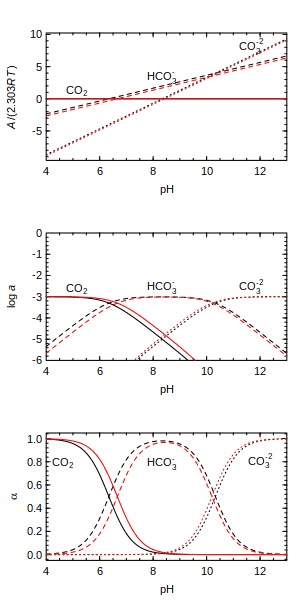Three views of carbonate speciation: affinity, activity, degree of formation.

The distribution of aqueous carbonate species as a function of pH (a type of Bjerrum plot) is a classic example of an equilibrium calculation. We can begin by plotting the affinities of formation, for equal activities of the species, calculated at 25 °C and 150 °C. Here, CO2 is in the basis, so it has zero affinity, which is greater than the affinities of HCO3- and CO3-2 at low pH. To avoid overplotting the lines, we offset the species labels in the y direction using the `dy` argument:

``````par(mfrow = c(3, 1))
basis("CHNOS+")
species(c("CO2", "HCO3-", "CO3-2"))
a25 <- affinity(pH = c(4, 13))
a150 <- affinity(pH = c(4, 13), T = 150)
diagram(a25, dy = 0.4)
diagram(a150, add = TRUE, names = NULL, col = "red")``````

Now we use `equilibrate()` to calculate the activities of species. Our balancing constraint is that the total activity of C is 10-3. This shows a hypothetical metastable equilibrium; we know that for true equilibrium the total activity of C is affected by pH.

``````e25 <- equilibrate(a25, loga.balance = -3)
e150 <- equilibrate(a150, loga.balance = -3)
diagram(e25, ylim = c(-6, 0), dy = 0.15)
diagram(e150, add = TRUE, names = NULL, col = "red")``````

To display the species distribution, or degree of formation, add `alpha = TRUE` to the argument list:

``````diagram(e25, alpha = TRUE, dy = -0.25)
diagram(e150, alpha = TRUE, add = TRUE, names = NULL, col = "red")``````

## Complete equilibrium: Solubility

It is important to remember that `equilibrate()` calculates an equilibrium distribution of species for a given total activity of the conserved basis species. For instance, the previous diagram shows the relative abundances of CO2, HCO3-, and CO3-2 as a function of pH assuming that the possible reactions between species are all balanced on 1 C and the total activity of C is constant. Although this assumption of metastable equilibrium is useful for making many types of diagrams for aqueous species, the aqueous solutions would not be in equilibrium with other phases, including gases or solids such as carbon dioxide or calcite.

Moving away from metastable equilibrium to complete equilibrium actually involves a simplification of the computations. Instead of finding the activities of aqueous species where the affinities of formation reactions are equal to each other, equilibrium corresponds to the configuration where the affinities are equal to zero. However, this simplification comes with a different constraint: the reactions should be balanced on something that anchors them to a real quantity. It makes sense to think of this quantity as a conserved basis species (one that is present in the formation reactions of all species considered), which corresponds to a pure substance that is being dissolved.

The `solubility()` function provides a way to compute activities of aqueous species in equilibrium with a solid or gas, which is usually defined as the first basis species. The following example for corundum (Al2O3) is based on Figure 15 of Manning (2013Manning CE. 2013. Thermodynamic modeling of fluid-rock interaction at mid-crustal to upper-mantle conditions. Reviews in Mineralogy and Geochemistry 76(1): 135–164. doi: 10.2138/rmg.2013.76.5). To reproduce the diagram, we use superseded thermodynamic data that are kept in the `SLOP98` optional data file. Corundum is set as the first basis species, and the formed species all contain Al. The affinities of the formation reactions, for unit activity of the solid basis species (corundum) and any given activities of the formed aqueous species, are input to `solubility()`. An additional argument, `in.terms.of`, is used to compute the total molality of Al in solution, that is, twice the number of moles of Al2O3 that are dissolved. `diagram()` is used twice, first to plot the total molality of Al, then the concentrations of the individual species, using `adj` and `dy` to adjust the positions of labels in the x- and y-directions. Note that setting `IS` to 0 in `affinity()` has no effect on the calculations, but signals `diagram()` to label the y axis with logarithm of molality instead of logarithm of activity. At the end of the calculation, we use `reset()` to restore the default thermodynamic database.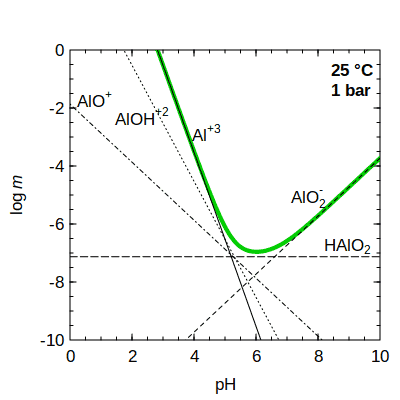Solubility of corundum (green line) and equilibrium concentrations of aqueous species (black lines).

``````add.obigt("SLOP98")
basis(c("corundum", "H2O", "H+", "O2"))
species(c("Al+3", "AlO2-", "AlOH+2", "AlO+", "HAlO2"))
a <- affinity(pH = c(0, 10), IS = 0)
s <- solubility(a, in.terms.of = "Al+3")
diagram(s, type = "loga.balance", ylim = c(-10, 0), lwd = 4, col = "green3")
diagram(s, add = TRUE, adj = c(0, 1, 2.1, -0.2, -1.5), dy = c(0, 0, 4, -0.3, 0.1))
legend("topright", c("25 °C", "1 bar"), text.font = 2, bty = "n")``````
``reset()``

Other examples of using `solubility()` are available in CHNOSZ. See `demo(solubility)` for calculations of the solubility of CO2(gas) and calcite as a function of pH and temperature. The calculation assumes the stoichiometric dissolution of calcite, in which CaCO3 dissociates to form equal quantities of Ca+2 and CO3-2 ions. Adding in activity coefficients, a different example in `?solubility` uses the `find.IS` option to find the final ionic strength for dissolving calcite into pure water. `demo(gold)` shows calculations of the solubility of gold as a function of pH and T as well as oxygen fugacity set by diferent mineral buffers, and considers ionic strength effects on activity coefficients, so that activities are transformed to molalities (see below).

## Groups of species

Sometimes it is helpful to look at the summed activities of species as groups on species distribution diagrams. The `groups` argument of `diagram()` can be used to sum the activities of species.

To demonstrate this feature, let’s consider the distribution of carbon among organic and inorganic species in the hydrothermal mixing scenario described by Shock and Schulte (1998Shock EL, Schulte MD. 1998. Organic synthesis during fluid mixing in hydrothermal systems. Journal of Geophysical Research 103(E12): 28513–28527. doi: 10.1029/98JE02142). First we define the basis and add two inorganic species. The `index.return = TRUE` argument tells `info()` to return the index (number) of the species in the current species definition; these indices are saved for use below:

``````basis("CHNOS+")
ii <- species(c("CO2", "HCO3-"), index.return = TRUE)``````

Next, we add each group of organic species: C1–C8 alcohols, C3–C8 ketones, C2–C12 carboxylic acids and their corresponding anions, and C2–C8 alkenes. To do this, we provide `info()` with a set of `ispecies` values that identify these species. In the database, the species in each group are ordered by carbon number, so we construct a sequence from the starting to ending `ispecies` for each group using R’s `seq()` function, wrapped by the `seq2()` function we write here to make the code shorter:

``````seq2 <- function(x) seq(x, x)
ia <- species(seq2(info(c("methanol", "octanol"))), index.return = TRUE)
ik <- species(seq2(info(c("acetone", "2-octanone"))), index.return = TRUE)
ic <- species(seq2(info(c("acetic acid","n-dodecanoic acid"))),index.return=TRUE)
ica <- species(seq2(info(c("acetate", "n-dodecanoate"))), index.return = TRUE)
ie <- species(seq2(info(c("ethylene", "octene"))), index.return = TRUE)``````

Now we read two data files that contain values of logfO2 and pH as a function of temperature, digitized from Figure 5 of Shock and Schulte (1998). The specific values are for calculations with vent fluids initially set by the fayalite-magnetite-quartz buffer minus 1/2 logfO2 (FMQ - 1/2). These values were calculated using a speciation and mixing model that is not available in CHNOSZ; however, we can use these intermediate values as input to the “downstream” calculations that are available in CHNOSZ. Because of the noise introduced by digitization of the figure, we smooth the data using R’s `smooth.spline()`; the lower T limit reflects the absence of data below this temperature in the figure for logfO2.

``````O2dat <- read.csv(system.file(
"extdata/cpetc/SS98_Fig5a.csv", package = "CHNOSZ"))
"extdata/cpetc/SS98_Fig5b.csv", package = "CHNOSZ"))
T <- seq(8, 350)
O2 <- predict(smooth.spline(O2dat\$T, O2dat\$logfO2), T)\$y
pH <- predict(smooth.spline(pHdat\$T, pHdat\$pH), T)\$y``````

We are ready to calculate affinities and equilibrium activities of the species. This calculation utilizes the transect mode of `affinity()` (see above). The call to `equilibrate()` runs with the default balance (in this case, CO2), with a log activity set to -2.5. Actually, the total concentration of carbon depends on the mixing ratio, ranging from about 10-2.2 (seawater) to 10-2.6 (vent fluid). A setting to vary the activity of the balanced basis species is not yet implemented in CHNOSZ, so a single value is used here.

``````a <- affinity(T = T, O2 = O2, pH = pH)
e <- equilibrate(a, loga.balance = -2.5)``````

At last we come to the diagram. The groups are identified by the current species numbers in a list; the elements of the list are given names that will appear on the diagram. When summing the activities of species in the groups, each activity is multiplied first by the balance coefficient on that species. Therefore, the total activity is that of a basis species (or of an element that is present only in that basis species, like carbon in this example).

``````par(mfrow = c(1, 3))
groups <- list(inorganic = ii, alcohols = ia, ketones = ik,
`carboxylic acids` = c(ic, ica), alkenes = ie)
diagram(e, alpha = TRUE, groups = groups, col = 1:5)``````

That makes a diagram that is similar to Figure 6b of Shock and Schulte (1998). Some differences from the original diagrams could be caused by the sensitivity of the calculations to logfO2, for which the values we use here may carry artifacts introduced by digitization of their plot. It is also possible to plot the distribution of species within individual groups, such as alcohols and ketones. We do this by setting the names and line types for the other species to values that prevent them from being plotted:

``````# plot only alcohols
names <- within(species(), name[-ia] <- "")\$name
lty <- ifelse(names == "", 0, 1)
diagram(e, alpha = TRUE, ylim = c(0, 0.32), lty = lty, names = names)
# plot only ketones
names <- within(species(), name[-ik] <- "")\$name
lty <- ifelse(names == "", 0, 1)
diagram(e, alpha = TRUE, ylim = c(0, 0.16), lty = lty, names = names)``````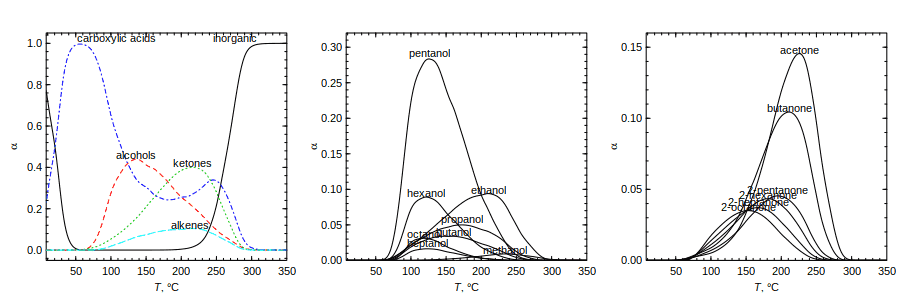Distribution of inorganic and groups of organic species (left plot) and of alcohols and ketones (middle and right plots) as a function of T, pH, and logfO2.

## Balancing differently

How about the choice between balancing constraints? Be default, `equilibrate()` and `diagram()` balance reactions on the first basis species that is present in each of the species of interest. Let’s look at some amino acids in a hypothetical metastable equilibrium. This calculation is loosely based on one described by Shock (1990Shock EL. 1990. Do amino acids equilibrate in hydrothermal fluids? Geochimica et Cosmochimica Acta 54(4): 1185–1189. doi: 10.1016/0016-7037(90)90450-Y) for five amino acids. Here we include 20 proteinogenic amino acids, whose names are returned by `aminoacids("")`. We use `ZC.col()` to generate colors based on the average oxidation state of carbon of the amino acids (red and blue for relatively reduced and oxidized).

``````basis("CHNOS")
basis("CO2", "gas")
swap.basis("NH3", "N2")
species(aminoacids(""))
a <- affinity(O2 = c(-50, -25, 200), CO2 = c(-10, 15, 200), T = 250, P = 265)
aa.ZC <- ZC(info(aminoacids("")))
col <- ZC.col(aa.ZC)``````

To make plots using different balance constraints, let’s write a function that sets the `balance` argument of `diagram()` and adds a title to the plot. The first plot is the most similar to Figure 4 of Shock (1990), except for the absence of alanine (probably due to different thermodynamic data) and the presence of some other amino acids. There, we set `balance = 1`, which indicates that moles of species are conserved; this is equivalent to balancing on the amino acid backbone. In the remaining plots, the balance is set to each of the basis species in turn (except for O2), then on volume. `expr.species()` together with R’s `substitute()` is used to make titles that include formatted chemical formulas:

``````aafun <- function(balance) {
diagram(a, balance = balance, fill = col)
blab <- expr.species(balance)
title(main = substitute("balanced on" ~ b, list(b = blab)))
}
par(mfrow = c(1, 5))
lapply(c("1", "CO2", "H2O", "N2", "volume"), aafun)``````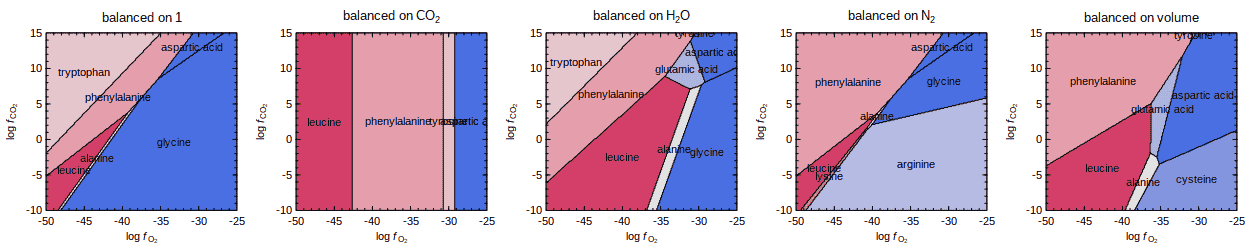Plots of maximum affinity at 250 °C and 265 bar using different reaction balances for 20 amino acids.

There are some broad similarities—increasing logfO2 favors more oxidized amino acids—but also substantial differences. It is interesting that there is more “going on” in the middle part of the diagram showing volume conservation.

Caveat lector. These plots demonstrate some possibilities in CHNOSZ and are not necessarily realistic portrayals of this system. It does seem odd to balance on a fugacious component like O2 or H2O. Unlike different choices of basis species, which are thermodynamically equivalent (see above), the choice of balance reflects extra-thermodynamic factors. For instance, the widespread rule of thumb for balancing mineral reactions on a chemical component is unrealistic for processes where volume is conserved (Merino and Dewers, 1998Merino E, Dewers T. 1998. Implications of replacement for reaction–transport modeling. Journal of Hydrology 209(1-4): 137–146. doi: 10.1016/S0022-1694(98)00150-4). While choosing an inappropriate balance leads to infeasible models, consideration of the different possibilities might give insight into the conditions affecting the dynamics of some systems.

# Activity coefficients

For calculating activity coefficients of charged species, `nonideal()` uses the extended Debye–Hückel equation as parameterized by Helgeson et al. (1981Helgeson HC, Kirkham DH, Flowers GC. 1981. Theoretical prediction of the thermodynamic behavior of aqueous electrolytes at high pressures and temperatures: IV. Calculation of activity coefficients, osmotic coefficients, and apparent molal and standard and relative partial molal properties to 600°C and 5 Kb. American Journal of Science 281(10): 1249–1516. doi: 10.2475/ajs.281.10.1249) for NaCl-dominated solutions to high pressure and temperature, or optionally using parameters described in Chapter 3 of Alberty (2003Alberty RA. 2003. Thermodynamics of Biochemical Reactions. Hoboken, New Jersey: Wiley-Interscience. Available at http://www.worldcat.org/oclc/51242181. ), which are applicable to relatively low-temperature biochemical reactions. The activity coefficients are calculated as a function of ionic strength (I), temperature, and charge of each species, without any other species-specific parameters. Using the default `Helgeson` method, the extended term parameter (“B-dot”) is derived from data of Helgeson (1969Helgeson HC. 1969. Thermodynamics of hydrothermal systems at elevated temperatures and pressures. American Journal of Science 267(7): 729–804. doi: 10.2475/ajs.267.7.729) and Helgeson et al. (1981), and extrapolations of Manning et al. (2013Manning CE, Shock EL, Sverjensky DA. 2013. The chemistry of carbon in aqueous fluids at crustal and upper-mantle conditions: Experimental and theoretical constraints. Reviews in Mineralogy and Geochemistry 75(1): 109–148. doi: 10.2138/rmg.2013.75.5).

## Transformation of variables

Following the main workflow of CHNOSZ, `nonideal()` normally does not need to be used directly. Intead, invoke the calculations by setting the `IS` argument in `subcrt()` or `affinity()`. There are a few things to remember when using activity coefficients:

• H+ is assumed to behave ideally, so its activity coefficient is 1 for any ionic strength. You can calculate activity coefficients of H+ by running `thermo("opt\$ideal.H" = FALSE)`.

• Using `subcrt()` with `IS` not equal to zero, calculated values of `G` are the adjusted Gibbs energy at specified ionic strength (denoted by ΔG°(I); Alberty, 1996Alberty RA. 1996. Recommendations for nomenclature and tables in biochemical thermodynamics. European Journal of Biochemistry 240(1): 1–14. Blackwell Science Ltd. doi: 10.1111/j.1432-1033.1996.0001h.x).

• Using `subcrt()` with `IS` not equal to zero, values in the `logact` argument stand for log molality of aqueous species in affinity calculations.

• Using `affinity()` with `IS` not equal to zero, the following values stand for log molality of aqueous species:
• values of `logact` set by `basis()`;
• values of `logact` set by `species()`.
• Using `equilibrate()` on the output of affinity calculated with `IS` not equal to zero, the following values stand for log molality of aqueous species:
• the value of `loga.balance` used by `equilibrate()` (i.e., logarithm of total molality of the balancing basis species);
• values of `loga.equil` returned by `equilibrate()`.

In other words, the activation of activity coefficients effects a transformation from activity to molality in the main workflow. A simple but comprehensive series of calculations demonstrating these tranformations is in `tests/testthat/test-logmolality.R`.

Because it is not possible to dynamically change the names of arguments in the functions, the user should be aware of the transformations mentioned above. However, the labels on diagrams can be automatically adjusted; accordingly, activities of aqueous species are relabeled as molalities by `diagram()` when `IS` is used in the calculation of `affinity()`.

## Biochemical example

For the following calculations, we change the nonideality method to `Alberty`; this is a simpler formulation with parameters that are suitable for biochemical species at relatively low temperatures:

``oldnon <- nonideal("Alberty")``
```## nonideal: setting nonideal option to use Alberty equation
```

Let’s take a look at calculated activity coefficients at two temperatures and their effect on the standard Gibbs energies of formation (ΔG°f) of species with different charge:

``````subcrt(c("MgATP-2", "MgHATP-", "MgH2ATP"),
T = c(25, 100), IS = c(0, 0.25), property = "G")\$out``````
```## subcrt: 3 species at 2 values of T and P (wet)
```
```## nonideal: calculations for MgC10H12N5O13P3-2, MgC10H13N5O13P3- (Alberty equation)
```
```## nonideal: calculations for MgC10H14N5O13P3 (Setchenow equation)
```
``````## \$`MgATP-2`
##     T       P       G    loggam   IS
## 1  25 1.00000 -773609  0.000000 0.00
## 2 100 1.01322 -780416 -0.656185 0.25
##
## \$`MgHATP-`
##     T       P       G    loggam   IS
## 1  25 1.00000 -780994  0.000000 0.00
## 2 100 1.01322 -789617 -0.164046 0.25
##
## \$MgH2ATP
##     T       P       G      loggam   IS
## 1  25 1.00000 -786200  0.00000000 0.00
## 2 100 1.01322 -795015 -0.00195159 0.25``````

The logarithms of the activity coefficients (`loggam`) are more negative for the higher-charged species, as well as at higher temperature, and have a stabilizing effect. That is, the adjusted Gibbs energies at I > 0 are less than the standard Gibbs energies at I = 0.

We can use these calculations to make some speciation plots, similar to Figures 1.2–1.5 in Alberty (2003). These figures show the distribution of differently charged species of adenosine triphosphate (ATP) as a function of pH, and the average number of H+ and Mg+2 bound to ATP in solution as a function of pH or pMg (-logaMg+2).

Use `info()` to see what ATP species are available. The sources of high-temperature thermodynamic data for these species are two papers by LaRowe and Helgeson (2006aLaRowe DE, Helgeson HC. 2006a. Biomolecules in hydrothermal systems: Calculation of the standard molal thermodynamic properties of nucleic-acid bases, nucleosides, and nucleotides at elevated temperatures and pressures. Geochimica et Cosmochimica Acta 70(18): 4680–4724. doi: 10.1016/j.gca.2006.04.010; 2006bLaRowe DE, Helgeson HC. 2006b. The energetics of metabolism in hydrothermal systems: Calculation of the standard molal thermodynamic properties of magnesium-complexed adenosine nucleotides and NAD and NADP at elevated temperatures and pressures. Thermochimica Acta 448(2): 82–106. doi: 10.1016/j.tca.2006.06.008).

``info(" ATP")``
```## info.approx: ' ATP' is ambiguous; has approximate matches to 14 species:
```
```##   "ATP-4"    "HATP-3"   "H2ATP-2"  "H3ATP-"   "H4ATP"    "dATP-4"
##   "dHATP-3"  "dH2ATP-2" "dH3ATP-"  "dH4ATP"   "MgATP-2"  "MgHATP-"
##  "MgH2ATP"  "Mg2ATP"
```

The plots for this system in Alberty’s book were made for I = 0.25 M and T = 25 °C. As a demonstration of CHNOSZ’s capabilities, we can assign a temperature of 100 °C.

``T <- 100``

Use the following commands to set the basis species, add the variously protonated ATP species, calculate the affinities of the formation reactions, equilibrate the system, and make a degree of formation (α) or mole fraction diagram. This is similar to Figure 1.3 of Alberty (2003), but is calculated for I = 0 M and T = 100 °C: To make the code more readable, commands for plotting titles and legends are not shown. All of the commands are available in the source of this document.

``````basis("MgCHNOPS+")
species(c("ATP-4", "HATP-3", "H2ATP-2", "H3ATP-", "H4ATP"))
a <- affinity(pH = c(3, 9), T = T)
e <- equilibrate(a)
d <- diagram(e, alpha = TRUE, tplot = FALSE)``````

Note that we have saved the numeric results of `diagram()`, i.e. the degrees of formation of the species (α). With that, we can calculate and plot the average number of protons bound per ATP molecule. To do so, we use R’s `rbind()` and `do.call()` to turn `alpha` into a matrix, then multiply by the number of protons bound to each species, and sum the columns to get the total (i.e. average proton number, NH+):

``````alphas <- do.call(rbind, d\$plotvals)
nH <- alphas * 0:4
Hlab <- substitute(italic(N)[H^`+`])
plot(a\$vals[], colSums(nH), type = "l", xlab = "pH", ylab=Hlab, lty=2, col=2)``````

Adding the `IS` argument to `affinity()`, we can now plot NH+ at the given ionic strength. Here we set `plot.it = FALSE` in `diagram()` because we use the computed α to make our own plot. This is similar to Figure 1.3 of Alberty (2003), but at higher temperature:

``````a <- affinity(pH = c(3, 9), IS = 0.25, T = T)
e <- equilibrate(a)
d <- diagram(e, alpha = TRUE, plot.it = FALSE)
alphas <- do.call(rbind, d\$plotvals)
nH <- alphas * 0:4
lines(a\$vals[], colSums(nH))``````

Next, we add the Mg+2-complexed ATP species:

``species(c("MgATP-2", "MgHATP-", "MgH2ATP", "Mg2ATP"))``

Here is a function to calculate and plot NH+ for a given pMg:

``````Hplot <- function(pMg, IS = 0.25) {
basis("Mg+2", -pMg)
a <- affinity(pH = c(3, 9), IS = IS, T = T)
e <- equilibrate(a)
d <- diagram(e, alpha = TRUE, plot.it = FALSE)
alphas <- do.call(rbind, d\$plotvals)
NH <- alphas * c(0:4, 0, 1, 2, 0)
lines(a\$vals[], colSums(NH), lty = 7 - pMg, col = 7 - pMg)
}``````

With that function in hand, we plot the lines corresponding to pMg = 2 to 6. This is similar to Figure 1.4 of Alberty (2003):

``````plot(c(3, 9), c(0, 2), type = "n", xlab = "pH", ylab = Hlab)
lapply(2:6, Hplot)``````

The next function calculates and plots the average number of Mg+2 bound to ATP (NMg+2) for a given pH. Here we multiply `alpha` by the number of Mg+2 in each species, and negate logaMg+2 (the variable used in `affinity()`) to get pMg:

``````Mgplot <- function(pH, IS = 0.25) {
basis("pH", pH)
a <- affinity(`Mg+2` = c(-2, -7), IS = IS, T = T)
e <- equilibrate(a)
d <- diagram(e, alpha = TRUE, plot.it = FALSE)
alphas <- do.call(rbind, d\$plotvals)
NMg <- alphas * species()\$`Mg+`
lines(-a\$vals[], colSums(NMg), lty = 10 - pH, col = 10 - pH)
}``````

Using that function, we plot the lines corresponding to pH = 3 to 9. This is similar to Figure 1.5 of Alberty (2003):

``````Mglab <- substitute(italic(N)[Mg^`+2`])
plot(c(2, 7), c(0, 1.2), type = "n", xlab = "pMg", ylab = Mglab)
lapply(3:9, Mgplot)``````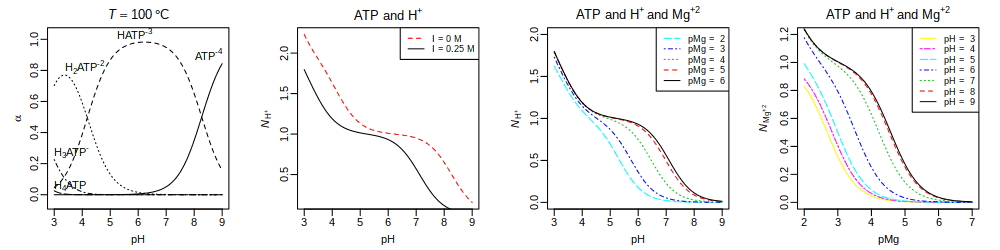Binding of H+ and Mg+2 to ATP at 100 °C and I = 0 M (first plot) or I = 0.25 M (third and fourth plots).

We have calculated the distribution of ATP species and average binding number of H+ and Mg+2 for given pH, pMg, ionic strength, and temperature. Accounting for the distribution of chemical species lends itself to thermodynamic models for reactions between reactants that have multiple ionized and complexed states. In contrast, Alberty (2003) and others propose models for biochemical reactions where the ionized and complexed species are combined into a single representation. Those models invoke Legendre-transformed thermodynamic properties, such as transformed Gibbs energies that are tabulated for specified pH, pMg, and ionic strength. Although the conceptual pathways are different, the two approaches lead to equivalent results concerning the energetics of the overall reactions and the conditions for equilibrium (Sabatini et al., 2012Sabatini A, Vacca A, Iotti S. 2012. Balanced biochemical reactions: A new approach to unify chemical and biochemical thermodynamics. PLoS ONE 7(1): e29529. doi: 10.1371/journal.pone.0029529). The example here shows how the required calculations can be performed at the species level using conventional standard Gibbs energies for species referenced to infinite dilution (zero ionic strength). The effects of ionic strength are modeled “on the fly” in CHNOSZ by setting the `IS` argument in `subcrt()` or `affinity()` to invoke the nonideality model on top of the standard Gibbs energies of species.

Now that we’re finished, we can reset the nonideality method to the default. (This really isn’t needed here, because there aren’t any nonideality calculations below):

``nonideal(oldnon)``
```## nonideal: setting nonideal option to use Bdot equation
```

# Proteins

Proteins in CHNOSZ are handled a little bit differently from other species. Amino acid group additivity is used to obtain the thermodynamic properties of proteins. Therefore, CHNOSZ has a data file with amino acid compositions of selected proteins, as well as functions for reading and downloading amino acid sequence data.

When proteins in CHNOSZ are identified by name, they include an underscore, such as in `LYSC_CHICK` (chicken lysozyme C). Use `pinfo()` to search for one or more proteins by name; multiple proteins from the same organism can be specified using the `organism` argument. The name search returns the rownumbers of `thermo()\$protein` (i.e. `iprotein`, the protein indices). Supply those protein indices to `pinfo()` to get the amino acid compositions:

``````p1 <- pinfo("LYSC_CHICK")
p2 <- pinfo(c("SHH", "OLIG2"), "HUMAN")
pinfo(c(p1, p2))``````
``````##     protein organism     ref    abbrv chains Ala Cys Asp Glu Phe Gly His Ile
## 6      LYSC    CHICK UniProt   P00698      1  12   8   7   2   3  12   1   6
## 452     SHH    HUMAN UniProt Q15465.N      1  14   3  10  14   5  16   6   7
## 453   OLIG2    HUMAN UniProt   Q13516      1  51   5  10  10   5  35  18   7
##     Lys Leu Met Asn Pro Gln Arg Ser Thr Val Trp Tyr
## 6     6   8   2  14   2   3  11  10   7   6   6   3
## 452  15  11   3   8   7   3  13  12   8   9   3   7
## 453  14  29  11   4  31   5  13  50  11  10   1   3``````

The length and chemical formula of one or more proteins are returned by `protein.length()` and `protein.formula()`. We can calculate the formula of the protein, and the per-residue formula, and show that both have the same average oxidation state of carbon: These functions accept either names or the protein indices (`iprotein`).

``````pl <- protein.length("LYSC_CHICK")
pf <- protein.formula("LYSC_CHICK")
list(length = pl, protein = pf, residue = pf / pl,
ZC_protein = ZC(pf), ZC_residue = ZC(pf / pl))``````
``````## \$length
##  129
##
## \$protein
##              C   H   N   O  S
## LYSC_CHICK 613 959 193 185 10
##
## \$residue
##                  C       H       N       O         S
## LYSC_CHICK 4.75194 7.43411 1.49612 1.43411 0.0775194
##
## \$ZC_protein
##  0.0163132
##
## \$ZC_residue
##  0.0163132``````

The group additivity calculations for proteins are based on equations and data from Amend and Helgeson (2000Amend JP, Helgeson HC. 2000. Calculation of the standard molal thermodynamic properties of aqueous biomolecules at elevated temperatures and pressures. II. Unfolded proteins. Biophysical Chemistry 84(2): 105–136. doi: 10.1016/S0301-4622(00)00116-2), Dick et al. (2006Dick JM, LaRowe DE, Helgeson HC. 2006. Temperature, pressure, and electrochemical constraints on protein speciation: Group additivity calculation of the standard molal thermodynamic properties of ionized unfolded proteins. Biogeosciences 3(3): 311–336. doi: 10.5194/bg-3-311-2006), and LaRowe and Dick (2012LaRowe DE, Dick JM. 2012. Calculation of the standard molal thermodynamic properties of crystalline peptides. Geochimica et Cosmochimica Acta 80: 70–91. doi: 10.1016/j.gca.2011.11.041). There are two major options for the calculations: whether to calculate properties for crystalline or aqueous groups, and, for the latter, whether to model the ionization of the sidechain and terminal groups as a function of pH (and T and P). By default, additivity of aqueous groups is used, but the ionization calculations are not available in `subcrt()`:

``subcrt("LYSC_CHICK")\$out[][1:6, ]``
``````##        T       P      rho    logK        G         H       S       V      Cp
## 1   0.01 1.00000 0.999829 3465.97 -4332111 -10713933 3846.41 10049.2 4409.32
## 2  25.00 1.00000 0.997061 3250.23 -4434110 -10573283 4337.86 10421.0 6415.52
## 3  50.00 1.00000 0.988030 3076.73 -4549372 -10403450 4884.51 10600.2 7073.98
## 4  75.00 1.00000 0.974864 2936.71 -4678266 -10222409 5423.99 10708.1 7376.58
## 5 100.00 1.01322 0.958393 2823.20 -4820386 -10035675 5941.89 10782.9 7548.44
## 6 125.00 2.32014 0.939073 2730.69 -4974814  -9845120 6435.26 10840.9 7665.20``````

Let’s compare experimental values of heat capacity of four proteins, from Privalov and Makhatadze (1990Privalov PL, Makhatadze GI. 1990. Heat capacity of proteins. II. Partial molar heat capacity of the unfolded polypeptide chain of proteins: Protein unfolding effects. Journal of Molecular Biology 213(2): 385–391. doi: 10.1016/S0022-2836(05)80198-6), with those calculated using group additivity. After dividing Privalov and Makhatadze’s experimental values by the lengths of the proteins to get per-residue values, we convert those to calories, then plot them.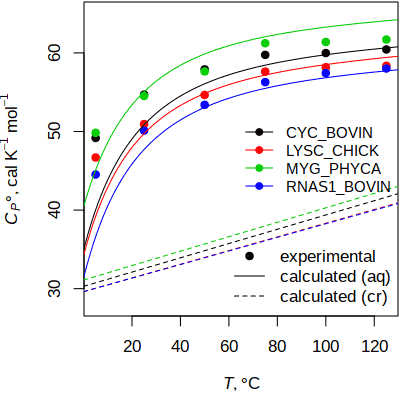The heat capacity calculated by group additivity closely approximates experimental values for aqueous proteins. For a related figure showing the effects of ionization in the calculations, see `?ionize.aa`.

``````PM90 <- read.csv(system.file("extdata/cpetc/PM90.csv", package = "CHNOSZ"))
plength <- protein.length(colnames(PM90)[2:5])
Cp_expt <- t(t(PM90[, 2:5]) / plength)
matplot(PM90[, 1], convert(Cp_expt, "cal"), type = "p", pch = 19,
xlab = axis.label("T"), ylab = axis.label("Cp0"), ylim = c(28, 65))``````

The loop calculates the properties of each protein using group additivity with aqueous or crystalline groups, then plots the per-residue values.

``````for(i in 1:4) {
pname <- colnames(Cp_expt)[i]
aq <- subcrt(pname, "aq", T = seq(0, 150))\$out[]
cr <- subcrt(pname, "cr", T = seq(0, 150))\$out[]
lines(aq\$T, aq\$Cp / plength[i], col = i)
lines(cr\$T, cr\$Cp / plength[i], col = i, lty = 2)
}
legend("right", legend = colnames(Cp_expt),
col = 1:4, pch = 19, lty = 1, bty = "n", cex = 0.9)
legend("bottomright", legend = c("experimental", "calculated (aq)",
"calculated (cr)"), lty = c(NA, 1, 2), pch = c(19, NA, NA), bty = "n")``````

Although `subcrt()` has no provision for protein ionization, the properties of ionization can be calculated via `affinity()`, which calls `ionize.aa()` if a charged species is in the basis. Whether to calculate properties using aqueous or crystalline groups is determined by the value of `thermo\$opt\$state`; if it is changed from its default of `aq` to `cr`, no ionization is possible. The following plot shows the calculated affinity of reaction between nonionized proteins and their ionized forms as a function of pH. Charged and uncharged sets of basis species are used to to activate and suppress the ionization calculations. The calculation of affinity for the ionized proteins returns multiple values (as a function of pH), but there is only one value of affinity returned for the nonionized proteins, so we need to use R’s `as.numeric()` to avoid subtracting nonconformable arrays: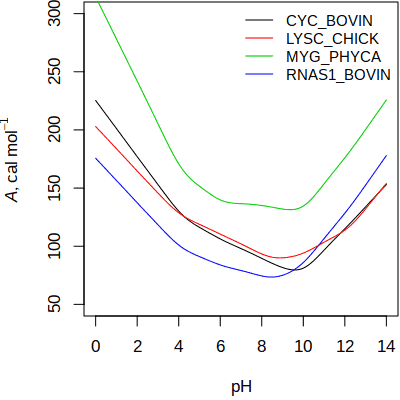Affinity of ionization of proteins. See demo(ionize) for ionization properties calculated as a function of temperature and pH.

``````ip <- pinfo(c("CYC_BOVIN", "LYSC_CHICK", "MYG_PHYCA", "RNAS1_BOVIN"))
basis("CHNOS+")
a_ion <- affinity(pH = c(0, 14), iprotein = ip)
basis("CHNOS")
a_nonion <- affinity(iprotein = ip)
plot(c(0, 14), c(50, 300), xlab = "pH", ylab = axis.label("A"), type = "n")
for(i in 1:4) {
A_ion <- as.numeric(a_ion\$values[[i]])
A_nonion <- as.numeric(a_nonion\$values[[i]])
lines(a_ion\$vals[], A_ion - A_nonion, col=i)
}
legend("topright", legend = a_ion\$species\$name,
col = 1:4, lty = 1, bty = "n", cex = 0.9)``````

The affinity is always positive, showing the strong energetic drive for ionization of proteins in aqueous solution. The degrees of ionization of amino and carboxyl groups increase at low and high pH, respectively, giving rise to the U-shaped lines.

There, we used the indices returned by `pinfo()` in the `iprotein` argument of `affinity()` to specify the proteins in the calculation. That approach utilizes some optimizations that can be realized due to group additivity, and is useful for calculations involving many proteins. An alternative, but slower, approach is to identify the proteins to `species()`; this produces results that are equivalent to using the `iprotein` argument:

``````species(c("CYC_BOVIN", "LYSC_CHICK", "MYG_PHYCA", "RNAS1_BOVIN"))
a_nonion_species <- affinity()``````
``unlist(a_nonion_species\$values)``
``````##      3362      3361      3365      3367
##   55.5242 -470.1077  228.5317 -495.8854``````

The `ispecies` index (top) refers to the rownumber of `thermo\$species`; that is distinct from the `iprotein` index (bottom, printed as negative integers), which refers to the rownumber of `thermo\$protein`.

``unlist(a_nonion\$values)``
``````##        -5        -6        -7        -9
##   55.5242 -470.1077  228.5317 -495.8854``````

## Compositional analysis

Functions in CHNOSZ make it easy to get the chemical formulas of proteins from their amino acid compositions. Calculations based on the formulas, such as the average oxidation state of carbon (ZC), and the coefficients of basis species in formation reactions, are also available.

Let’s compare the ZC of Rubisco with optimal growth temperature of organisms, as shown in Figure 6a of Dick (2014Dick JM. 2014. Average oxidation state of carbon in proteins. Journal of the Royal Society Interface 11: 20131095. doi: 10.1098/rsif.2013.1095). First we read a CSV file with the IDs of the proteins and the optimal growth temperatures (Topt); the midpoint of the range of Topt is used for plotting. Then we use `read.fasta()` to read a FASTA file holding the amino acid sequences of the proteins; the function returns a data frame with the amino acid counts. To put the proteins in the right order, the IDs in the CSV file are matched to the names of the proteins in the FASTA file. Then, we calculate ZC from the formulas of the proteins. Next, point symbols are assigned according to domain (Archaea, Bacteria, Eukaryota); numbers inside the symbols show the ordering of Topt in three temperature ranges (0–35 °C, 37.5–60 °C, and 65–100 °C).

Average oxidation state of carbon in Rubisco compared with optimal growth temperature of organisms. This is an interactive image. Move the mouse over the points to show the names of the organisms, and click to open a reference in a new window. (Made with RSVGTipsDevice using code that can be found in the source of this document.)

``````datfile <- system.file("extdata/cpetc/rubisco.csv", package = "CHNOSZ")
fastafile <- system.file("extdata/fasta/rubisco.fasta", package = "CHNOSZ")
Topt <- (dat\$T1 + dat\$T2) / 2
idat <- match(dat\$ID, substr(aa\$protein, 4, 9))
aa <- aa[idat, ]
ZC <- ZC(protein.formula(aa))
pch <- match(dat\$domain, c("E", "B", "A")) - 1
col <- match(dat\$domain, c("A", "B", "E")) + 1
plot(Topt, ZC, pch = pch, cex = 2, col = col,
xlab = expression(list(italic(T)[opt], degree*C)),
ylab = expression(italic(Z)[C]))
text(Topt, ZC, rep(1:9, 3), cex = 0.8)
abline(v = c(36, 63), lty = 2, col = "grey")
legend("topright", legend = c("Archaea", "Bacteria", "Eukaryota"),
pch = c(2, 1, 0), col = 2:4, pt.cex = 2)``````

Let’s look at the different ways of representing the chemical compositions of the proteins. `protein.basis()` returns the stoichiometry for the formation reaction of each proteins. Dividing by `protein.length()` gives the per-residue reaction coefficients (n̅). Using the set of basis species we have seen before (CO2, NH3, H2S, H2O, O2) there is a noticeable correlation between n̅O2 and ZC, but even more so between n̅H2O and ZC (shown in the plots on the left). The calculation of ZC, which sums elemental ratios, is not affected by the choice of basis species. The `QEC` keyword to `basis()` loads basis species including three amino acids (glutamine, glutamic acid, cysteine, H2O, O2). This basis strengthens the relationship between ZC and n̅O2, but weakens that between ZC and n̅H2O (shown in the plots on the right).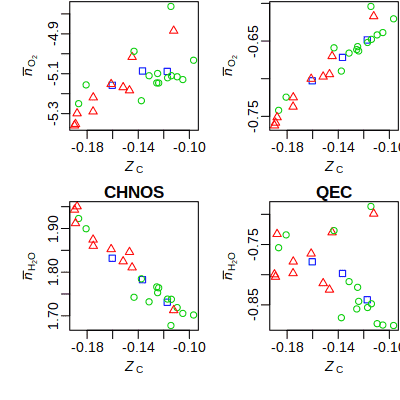Compositions of proteins projected into different sets of basis species.

``````layout(matrix(1:4, nrow = 2))
par(mgp = c(1.8, 0.5, 0))
pl <- protein.length(aa)
ZClab <- axis.label("ZC")
nO2lab <- expression(bar(italic(n))[O])
nH2Olab <- expression(bar(italic(n))[H*O])
lapply(c("CHNOS", "QEC"), function(thisbasis) {
basis(thisbasis)
pb <- protein.basis(aa)
nO2 <- pb[, "O2"] / pl
plot(ZC, nO2, pch = pch, col = col, xlab = ZClab, ylab = nO2lab)
nH2O <- pb[, "H2O"] / pl
plot(ZC, nH2O, pch = pch, col = col, xlab = ZClab, ylab = nH2Olab)
mtext(thisbasis, font = 2)
})``````

By projecting the compositions of proteins into the `QEC` set of basis species, n̅O2 emerges as a strong indicator of oxidation state, while n̅H2O is a relatively uncorrelated (i.e. independent) compositional variable. These independent variables make it easier to distinguish the effects of oxidation and hydration state in proteomic datasets (Dick, 2017Dick JM. 2017. Chemical composition and the potential for proteomic transformation in cancer, hypoxia, and hyperosmotic stress. PeerJ 5: e3421. doi: 10.7717/peerj.3421).

## Normalization to residues

As with other systems, a balance must be chosen for calculations of the metastable equilibrium distribution for proteins. Balancing on the number of backbone units (the sequence length) seems a reasonable choice given the polymeric structure of proteins. Balancing on one of the basis species remains a possibility, using the `balance` argument in `equilibrate()` or `diagram()`. However, there is an additional consideration: owing to the large size of proteins compared to the basis species, the distribution of proteins in metastable equilibrium has many orders of magnitude separation between the activities of the dominant and less-dominant proteins. The metastable coexistence of the residues (i.e. per-residue formulas, or residue equivalents) of the same proteins spans a much smaller range of chemical activities. In CHNOSZ, the calculation of metastable equilibrium activities of the residue equivalents is referred to as normalization. See the vignette Equilibrium in CHNOSZ for other examples using normalization.

To take an example, let’s look at the metastable equilibrium distribution of selected proteins in the ER-to-Golgi location of S. cerevisiae (yeast). This example brings in a few other functions we haven’t seen yet: `yeastgfp()`, `unitize()`, and `revisit()`. `yeastgfp()` queries a data file in CHNOSZ for the names and relative abundances of proteins taken from the YeastGFP study of Ghaemmaghami et al. (2003Ghaemmaghami S, Huh W-K, Bower K, Howson RW, Belle A, Dephoure N, O’Shea EK, Weissman JS. 2003. Global analysis of protein expression in yeast. Nature 425(6959): 737–741. doi: 10.1038/nature02046). There are six proteins identified in the ER-to-Golgi location; one has NA abundance, so it is excluded from the comparisons:

``````y <- yeastgfp("ER.to.Golgi")
ina <- is.na(y\$abundance)``````

Next, we get the amino acid compositions of the proteins and add them to `thermo()\$protein`:

``````aa <- yeast.aa(y\$protein[!ina])

The YeastGFP study reported absolute abundances of molecules, but the thermodynamic calculations give relative chemical activities of the proteins. In order to make a comparison between them, we use `unitize()` to scale the abundances or activities of proteins (in logarithmic units) such that the total abundance or activity of residue equivalents is unity. To do that, we must have the lengths of the proteins. Here, the first call to `unitize()` generates equal logarithms of activities of proteins for unit total activity of residues; this is used as the reference state for `affinity()`. The second call to `unitize()` scales the logarithms of experimental abundances for unit total activity of residues; this is used for comparison with the theoretical results:

``````pl <- protein.length(ip)
logact <- unitize(numeric(5), pl)
logabundance <- unitize(log10(y\$abundance[!ina]), pl)``````

Now we can load the proteins and calculate their activities in metastable equilibrium as a function of logfO2. The commented line uses `add.obigt()` to load group additivity parameters that were present in older versions of CHNOSZ (Dick et al., 2006). The default database contains newer group additivity parameters for the sidechain groups of methionine (LaRowe and Dick, 2012) and glycine and the protein backbone (Kitadai, 2014Kitadai N. 2014. Thermodynamic prediction of glycine polymerization as a function of temperature and pH consistent with experimentally obtained results. Journal of Molecular Evolution 78(3-4): 171–187. doi: 10.1007/s00239-014-9616-1).

``````par(mfrow = c(1, 3))
basis("CHNOS+")
a <- affinity(O2 = c(-80, -73), iprotein = ip, loga.protein = logact)
e <- equilibrate(a)
diagram(e, ylim = c(-5, -2), col = 1:5, lwd = 2)``````

Whoa! The proteins look very non-coexistent in metastable equilibrium. We get a different view by considering per-residue rather than per-protein reactions, through the `normalize` argument for `equilibrate()`: The normalization step is followed by conversion of activities of residues to activities of proteins; that conversion can be skipped using the `as.residue` argument in `equilibrate()`.

``````e <- equilibrate(a, normalize = TRUE)
diagram(e, ylim = c(-5, -2.5), col = 1:5, lwd = 2)
abline(h = logabundance, lty = 1:5, col = 1:5)``````

The experimental relative abundances are plotted as thin horizontal lines with the same style and color as the thicker curved lines calculated for metastable equilibrium. With the exception of YNL049C, the overlap between the calculations and experiments looks to be greatest near the middle-left part of the figure. The `revisit()` function (see below) offers some statistical and thermodynamic measures that can quantify this comparison. Here, we plot the information-theoretic free energy ΔGinf (calculated from the relative entropy or Kullback–Leibler divergence):

``revisit(e, "DGinf", logabundance)``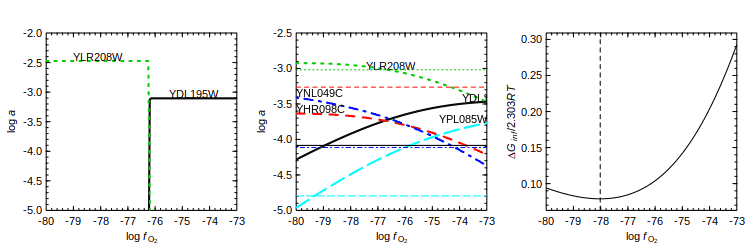ER-to-Golgi proteins: calculations without and with length normalization, and free energy difference between experimental and calculated abundances in metastable equilibrium with normalization.

The minimum free energy difference occurs near logfO2 = -78. This agrees with the assessment shown in Figure 4 of Dick (2009Dick JM. 2009. Calculation of the relative metastabilities of proteins in subcellular compartments of saccharomyces cerevisiae. BMC Systems Biology 3: 75. doi: 10.1186/1752-0509-3-75) (but the updated group additivity parameters make the results slightly different).

## An affinity baseline

Because affinities of proteins often vary strongly with oxygen fugacity and other variables, it can be helpful to express the values as differences from a baseline. The following example compares the affinities for formation of transcription factors involved in embryonic dorsal-ventral patterning with that of the morphogen, Sonic hedgehog (Shh), as a function of logfO2 and logaH2O (Dick, 2015Dick JM. 2015. Chemical integration of proteins in signaling and development. bioRxiv. doi: 10.1101/015826). We first list the UniProt names of Shh and 10 transcription factors, and get the `iprotein` indices (rownumbers of `thermo()\$protein`):

``````pname <- c("SHH", "OLIG2", "NKX22", "FOXA2", "IRX3",
"PAX6", "NKX62", "DBX1", "DBX2", "NKX61", "PAX7")
ip <- pinfo(pname, "HUMAN")``````

Next, set up the basis species:

``````basis("CHNOS")
basis("NH3", -7)``````

Now calculate the affinities of formation of the proteins from the basis species. The values chosen for logfO2 and logaH2O covary, so we are using the transect mode of `affinity()`:

``````O2 <- seq(-70, -106, length.out = 50)
H2O <- seq(0.5, -5.5, length.out = 50)
a <- affinity(H2O = H2O, O2 = O2, iprotein = ip)``````

We would like to compare the affinities of the proteins on a per-residue scale. R’s `lapply()` could be used here, but to show the operation more clearly we use a `for()` loop:

``````pl <- protein.length(ip)
for(i in seq_along(a\$values)) a\$values[[i]] <- a\$values[[i]] / pl[i]``````

Then, we calculate the relative affinities, using Shh as the baseline:

``````a.Shh <- a\$values[]
for(i in 1:length(a\$values)) a\$values[[i]] <- a\$values[[i]] - a.Shh``````

Next we use `diagram()` to plot the affinities. We set `balance = 1` to plot the values as they are—without that, `diagram()` divides the values by protein length, which we have already done! See `demo(Shh)` for a plot with more interpretive labels and comments. For this plot, we highlight and label the proteins with the highest relative affinity at some combination of logfO2 and logaH2O along the transect. Those proteins are Olig2, Irx3, Nkx6.2, Dbx1, and Shh (numbers 2, 5, 7, 8, 1 in the set we have identified). Here, `adj = 0` makes the labels left-aligned, `dy = 0.1` adds a y offset to the labels, and `format.names = FALSE` prevents formatting of the names as if they were chemical formulas (that causes subscripted numbers to appear). The last few lines are used to make a second x axis, using a label generated with `axis.label()`: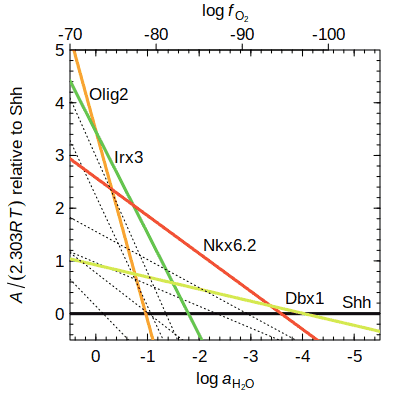Per-residue affinities for formation of transcription factors relative to Shh.

``````# line type, width, and color
twc <- lapply(c(3, 1, 1), rep, length(pname))
ihigh <- c(2, 5, 7, 8, 1)
twc[][ihigh] <- 1
twc[][ihigh] <- 3
col <- c("#f9a330", "#63c54e", "#f24e33", "#d4e94e", "#0f0f0f")
twc[][ihigh] <- col
names <- rep("", length(pname))
names[ihigh] <- c("Olig2", "Irx3", "Nkx6.2", "Dbx1", "Shh")
ylab <- substitute(italic(A) / (2.303 * italic(RT)) * " relative to Shh")
diagram(a, balance = 1, ylim = c(-0.5, 5), xlim = c(0.5, -5.5),
lty = twc[], lwd=twc[], col = twc[], ylab = ylab,
names = names, adj = 0, dy = 0.1, format.names = FALSE)
par(usr = c(-70, -106, -0.5, 5), tcl = -0.3)
axis(3, at = seq(-70, -106, by = -10))
mtext(axis.label("O2"), line = 1.2)``````

Among these proteins, Olig2 and Shh have the greatest affinities for formation at the extremes of oxidation and hydration state. This thermodynamic description highlights possible links between the chemical compositions of the proteins and their patterns of expression along with chemical changes in developing embryos (Dick, 2015).

## Getting amino acid compositions

In the Rubisco example above, we saw the use of `read.fasta()` to read amino acid sequences from a FASTA file. There are several other methods for inputting amino acid compositions.

R’s `read.csv()` can be used to read amino acid compositions from a CSV file with the same columns that are present in `thermo()\$protein`. Note the use of `as.is = TRUE` to prevent reading character data as factors. The `nrows` argument can be added to read that number of rows:

``````file <- system.file("extdata/protein/DS11.csv", package = "CHNOSZ")
aa_bison <- read.csv(file, as.is = TRUE, nrows = 5)``````

`yeast.aa()` retrieves amino acid composition of proteins in Saccharomyces cerevisiae from a data file that is included with CHNOSZ:

``aa_YML020W <- yeast.aa("YML020W")``

`read.fasta()` reads a FASTA file and returns the amino acid compositions of the sequences. The `iseq` argument can be used to read those sequences from the file:

``````file <- system.file("extdata/fasta/EF-Tu.aln", package = "CHNOSZ")
aa_Ef <- read.fasta(file, iseq = 1:2)``````

`seq2aa()` counts the amino acids in a user-supplied sequence and generates a data frame of the amino acid composition: See also `?count.aa`, which can process both protein and nucleic acid sequences.

``````aa_PRIO <- seq2aa("PRIO_HUMAN", "
MANLGCWMLVLFVATWSDLGLCKKRPKPGGWNTGGSRYPGQGSPGGNRYPPQGGGGWGQP
HGGGWGQPHGGGWGQPHGGGWGQPHGGGWGQGGGTHSQWNKPSKPKTNMKHMAGAAAAGA
VVGGLGGYMLGSAMSRPIIHFGSDYEDRYYRENMHRYPNQVYYRPMDEYSNQNNFVHDCV
NITIKQHTVTTTTKGENFTETDVKMMERVVEQMCITQYERESQAYYQRGSSMVLFSSPPV
ILLISFLIFLIVG
")``````

`uniprot.aa()` returns the amino acid composition of a single amino acid sequence downloaded from UniProt. To get sequences for many proteins, use R’s `lapply()`, `do.call()`, and `rbind()`:

``````IDs <- c("ALAT1_HUMAN", "P02452")
aa <- lapply(IDs, uniprot.aa)
## uniprot.aa: trying http://www.uniprot.org/uniprot/ALAT1_HUMAN ... accession P24298 ...
## >sp|P24298|ALAT1_HUMAN Alanine aminotransferase 1 OS=Homo sapiens GN=GPT PE=1 SV=3 (length 496)
## uniprot.aa: trying http://www.uniprot.org/uniprot/P02452 ... accession P02452 ...
## >sp|P02452|CO1A1_HUMAN Collagen alpha-1(I) chain OS=Homo sapiens GN=COL1A1 PE=1 SV=5 (length 1464)
aa_UniProt <- do.call(rbind, aa)``````
``aa_UniProt``
``````##      protein organism ref abbrv chains Ala Cys Asp Glu Phe Gly His Ile Lys Leu
## 1  sp|P24298    HUMAN  NA ALAT1      1  51  10  20  34  21  38   9  18  16  55
## 11 sp|P02452    HUMAN  NA CO1A1      1 139  18  66  75  27 391   9  24  57  48
##    Met Asn Pro Gln Arg Ser Thr Val Trp Tyr
## 1   12  12  33  30  37  25  18  41   1  15
## 11  13  28 278  49  71  60  45  47   6  13``````

These amino acid compositions can be processed using functions such as `protein.length()` and `protein.formula()`:

``````myaa <- rbind(aa_YML020W, aa_Ef, aa_PRIO)
protein.length(myaa)``````
``##  664 394 394 253``

## Adding proteins and using `iprotein`

Once the amino acid compositions have been obtained, use `add.protein()` to add these proteins to `thermo()\$protein`:

``add.protein(myaa)``
```## add.protein: added 4 new protein(s) to thermo\$protein
```
``##  512 513 514 515``

Then, `subcrt()` can be used to calculate the standard thermodynamic properties of any of these proteins:

``subcrt("PRIO_HUMAN", T = 25)``
``````## \$species
##            name               formula state ispecies
## 3390 PRIO_HUMAN C1227H1834N352O352S16    aq     3390
##
## \$out
## \$out\$PRIO_HUMAN
##    T P      rho    logK        G         H       S       V      Cp
## 1 25 1 0.997061 5903.69 -8054078 -19645677 8412.06 20084.3 12174.4``````

Or we can add any of these proteins to the species list with `species()` and calculate the affinity:

``basis("CHNOS")``
``species("YML020W_Sce")``
``````##    CO2  H2O NH3 H2S    O2 ispecies logact state        name
## 1 3406 1247 932  13 -3517     3391     -3    aq YML020W_Sce``````
``a <- affinity()``

Or we can calculate the affinities of formation reactions of the proteins without adding them as species:

``````ip <- add.protein(aa_UniProt)
a <- affinity(iprotein = ip)``````

As shown there, the `iprotein` argument of `affinity()` can be used to calculate the affinities of reactions to form the indicated proteins, bypassing the `species()` step. Let’s see this in action using amino acid compositions deduced from metagenomic sequences in the Bison Pool hot spring in Yellowstone (Dick and Shock, 2011Dick JM, Shock EL. 2011. Calculation of the relative chemical stabilities of proteins as a function of temperature and redox chemistry in a hot spring. PLOS One 6(8): e22782. doi: 10.1371/journal.pone.0022782). We read a data file of amino acid compositions produced in that study, taking those labeled “transferase”. Then we add the proteins and get their indices using `add.protein()`, set the basis, calculate the affinities, and make a potential diagram with temperature and activity of dissolved hydrogen as variables: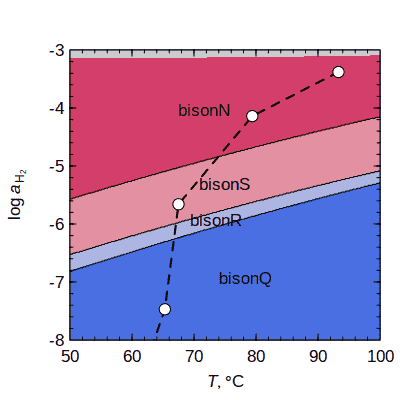Potential diagram for metagenomically identified sequences of transferases in Bison Pool hot spring. See also the vignette Hot-spring proteins in CHNOSZ.

``````file <- system.file("extdata/protein/DS11.csv", package = "CHNOSZ")
aa <- read.csv(file, as.is = TRUE)
aa <- aa[grep("transferase", aa\$protein), ]
basis(c("HCO3-", "H2O", "NH3", "HS-", "H2", "H+"))
basis(c("HCO3-", "NH3", "HS-", "H+"), c(-3, -4, -7, -7.9))
T <- c(50, 100)
res <- 300
a <- affinity(T = c(T, res), H2 = c(-8, -3, res), iprotein = ip)
fill <- ZC.col(ZC(protein.formula(ip)))
diagram(a, normalize = TRUE, fill = fill, names = 1:5)``````

Site numbers 1–5 correspond to a cooling gradient along the outflow channel of the hot spring. The colors represent the relative ZC of the proteins (red is more reduced). The points indicate the T and logaH2 that optimize a thermodynamic model for relative abundances of phyla that were estimated by taxonomic classification of metagenomic sequences (Dick and Shock, 2013Dick JM, Shock EL. 2013. A metastable equilibrium model for the relative abundances of microbial phyla in a hot spring. PLOS One 8(9): e72395. doi: 10.1371/journal.pone.0072395):

``````T <- c(93.3, 79.4, 67.5, 65.3, 57.1)
logaH2 <- c(-3.38, -4.14, -5.66, -7.47, -10.02)
lines(T, logaH2, lty = 2, lwd = 2)
points(T, logaH2, pch = 21, bg = "white", cex = 1.5)``````

# Optimization of chemical activities

NOTE: This is an experimental feature.

What are the conditions that minimize the standard deviation of calculated activities of species? What are the conditions that minimize the energetic difference between measured and calculated abundances? These are questions about optimization of chemical activities. `revisit()` provides calculations and plots of an objective function in 1 or 2 dimensions (activities of basis species, T, or P). See `?objective` for more information. `findit()` provides a calculation of an objective function on a higher-dimensional grid.

The concentrations of amino acids are affected by high-temperature reactions in hydrothermal vents (smokers). What are the metastable equilibrium states of amino acids under these conditions? Suppose that the major variables are oxygen fugacity, and activities of water (H2O) and ammonia (NH3). Here we assign the (very large) range of logarithms of chemical activity of the basis species that we will explore:

``vars <- list(O2 = c(-50, -25), NH3 = c(-15, 10), H2O = c(-15, 10))``

Consider the amino acid abundances reported by Fuchida et al. (2014Fuchida S, Mizuno Y, Masuda H, Toki T, Makita H. 2014. Concentrations and distributions of amino acids in black and white smoker fluids at temperatures over 200 °C. Organic Geochemistry 66: 98–106. doi: 10.1016/j.orggeochem.2013.11.008). Here, we identify the amino acids using their one-letter abbreviations. Then, `aminoacids()` is used to produce the full names, which in turn are used to search `thermo()\$obigt` for their formulas. `makeup()` is used to count the elements in the formulas:

``````aa <- c("D", "T", "S", "E", "G", "A", "K", "H")
AA <- aminoacids("", aa)
AA.formula <- do.call(rbind, makeup(info(AA)))
AA``````
``````##  "aspartic acid" "threonine"     "serine"        "glutamic acid"
##  "glycine"       "alanine"       "lysine"        "histidine"``````

Let’s use the reported concentrations (µM) of amino acids in sample D1441 CW-2. Three amino acids (Val, Phe, Arg) with concentrations below the detection limit of 0.01 µM are not included here. The concentrations are converted to logarithmic values (`loga2`). We then calculate the total C concentration in the measurements; this uses the amino acid formulas calculated above:

``````uM <- c(1.10, 0.70, 3.73, 0.39, 3.04, 1.83, 0.27, 0.21)
loga2 <- log10(uM * 1e-6)
nC <- AA.formula[, "C"]
Ctot <- sum(nC * uM * 1e-6)``````

Next, we set the basis, load the species, and assign the temperature and resolution to be used in `affinity()`. Also, we assign the objective function to be the root mean square deviation (RMSD) between experimental and calculated logarithms:

``````basis("CHNOS")
species(AA)
T <- 270
res <- 64
objective <- "RMSD"``````

Then, we set up the figure, calculate chemical affinities in one dimension, and run `equilibrate()` with the given total carbon activity. `revisit()` is used to plot RMSD and to identify the logfO2 where it is minimized:

``````layout(matrix(1:6, nrow = 2))
a <- affinity(O2 = c(vars[], res), T = T)
e <- equilibrate(a, loga.balance = log10(Ctot))
r <- revisit(e, objective, loga2)
d.basis <- describe.basis(ibasis = 1:4)
legend("topleft", d.basis)``````

Now, we write a function that calculates the affinities and metastable equilibrium activities at a single condition and uses `revisit()` to make a scatter plot. The plot includes a 1:1 line (grey), a trend line calculated using R’s `loess.smooth()` (red), and a title with the minimum value of RMSD. The function also adds a legend summarizing the optimal conditions:

``````ourfun <- function(ibasis = 1:5, x = "bottomright") {
a <- affinity(T = T)
e <- equilibrate(a, loga.balance = log10(Ctot))
revisit(e, objective, loga2, cex = 2.7, pch = 21)
text(loga2, unlist(e\$loga.equil), aa)
d.basis <- describe.basis(ibasis = ibasis)
legend(x, d.basis)
}``````

We set the activity of logfO2 in the basis (where RMSD was minimized the first call to `revisit()`), then use our function to make a scatter plot:

``````basis("O2", r\$xopt)
ourfun(5)``````

In the next pair of plots, let’s use two variables: logfO2 and logaNH3. This time, `revisit()` makes a contour diagram of the objective function, highlighting the location of the minimum (star) as well as the minimum trough following the x or y axis (dotted blue and green lines). After that, we set the activities of both of the basis species and then use `ourfun()` to make a scatter plot. The legend here shows the two variables that have been optimized; these conditions yield an RMSD that is lower than in the first calculation:

``````a <- affinity(O2 = c(vars[], res), NH3 = c(vars[], res), T = T)
e <- equilibrate(a, loga.balance = log10(Ctot))
r <- revisit(e, objective, loga2)
basis("O2", r\$xopt)
basis("NH3", r\$yopt)
ourfun(c(3, 5))``````

Finally, we use `findit()` to optimize three variables. The affinity and equilibrium calculations are integrated into `findit()`, so the function call requires the `loga.balance` and `T` arguments. Other arguments indicate the grid resolution, factor (ratio) by which variable ranges are reduced in each step, and the number of iterations. The result is a series of 2D contour plots of decreasing range, each one constructed for the optimal value of logaH2O from the previous step. More dimensions require a lower resolution due to limited computational resources. While running, `findit()` sets the activities of basis species, so we can immediately call `ourfun()` to make make a scatter plot. This side effect (changing the activities of basis species) is why the text for `findit()` is colored red.

``````findit(vars[1:3], objective, loga2 = loga2, loga.balance = log10(Ctot),
T = T, res = 8, niter = 3, rat = 0.6)
ourfun(c(2, 3, 5), "right")``````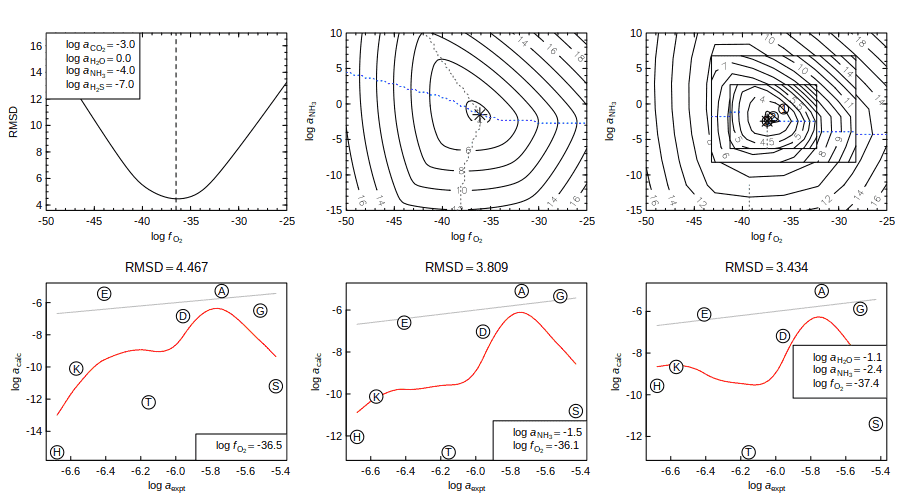Optimization of a thermodynamic model for relative abundances of amino acids in a 270 °C black smoker fluid using 1, 2, or 3 variables (left to right).

The calculation using `findit()`, in which the added variable logaH2O optimizes to ca. -2.4, shows that measured concentrations of 6 amino acids fall within 1–2 log units of the relative abundances in metastable equilibrium. According these calculations, two amino acids, serine and threonine, are very far from metastable equilibrium with the others. There is a danger of using too many, or unrepresentative variables, but in some systems, calculations of this type may provide insight on processes affecting reactions of organic compounds.

# Thermodynamic data

## Viewing data sources: `thermo.refs()`

The database in CHNOSZ lists one or two sources for each entry, and citation information for the sources is listed in `thermo()\$refs`. You can locate and view the references with `thermo.refs()`. Running the function without any arguments opens a browser window with the complete table of references. See the vignette, Thermodynamic data in CHNOSZ, for a more nicely formatted presentation of the sources of thermodynamic data, along with notes and additional comments. Where available, links to the web page for the articles and books are displayed:

``thermo.refs()  ## shows table in a browser``

A numeric argument to `thermo.refs()` gives one or more species indices for which to get the references:

``````iATP <- info("ATP-4")
iMgATP <- info("MgATP-2")
thermo.refs(c(iATP, iMgATP))``````
``````##       key                          author year                               citation                                                    note
## 134 LH06a D. E. LaRowe and H. C. Helgeson 2006 Geochim. Cosmochim. Acta 70, 4680-4724        nucleic-acid bases, nucleosides, and nucleotides
## 136 LH06b D. E. LaRowe and H. C. Helgeson 2006           Thermochim. Acta 448, 82-106 Mg-complexed adenosine nucleotides (ATP), NAD, and NADP
##                                           URL
## 134 https://doi.org/10.1016/j.gca.2006.04.010
## 136 https://doi.org/10.1016/j.tca.2006.06.008``````

A character argument gives the source key(s):

``thermo.refs(c("HDNB78", "MGN03"))``
``````##        key                                    author year                 citation                                       note                                   URL
## 7   HDNB78       H. C. Helgeson, J. M. Delany et al. 1978  Am. J. Sci. 278A, 1-229    data for minerals and phase transitions http://www.worldcat.org/oclc/13594862
## 123  MGN03 J. Majzlan, K.-D. Grevel and A. Navrotsky 2003 Am. Mineral. 88, 855-859 goethite, lepidocrocite, and maghemite GHS https://doi.org/10.2138/am-2003-5-614``````

If the argument holds the result of `subcrt()`, references for all species in the reaction are returned: The exception is H2O. With the default settings, thermodynamic properties for H2O are derived from SUPCRT92 (Johnson et al., 1992).

``````substuff <- subcrt(c("C2H5OH", "O2", "CO2", "H2O"), c(-1, -3, 2, 3))
thermo.refs(substuff)``````
``````##       key                                           author year                               citation                      note                                           URL
## 113  PS01                  A. V. Plyasunov and E. L. Shock 2001 Geochim. Cosmochim. Acta 65, 3879-3900   aqueous nonelectrolytes https://doi.org/10.1016/S0016-7037(01)00678-0
## 30  SHS89 E. L. Shock, H. C. Helgeson and D. A. Sverjensky 1989 Geochim. Cosmochim. Acta 53, 2157-2183 inorganic neutral species  https://doi.org/10.1016/0016-7037(89)90341-4``````

The URLs of the references can be copied to a browser, or opened using R’s `browseURL()`:

``````iFo <- info("forsterite")
ref <- thermo.refs(iFo)
browseURL(ref\$URL)  ## opens a link to worldcat.org``````

## Optional data

Thermodynamic properties of minerals in the default database are mostly taken from Berman (1988Berman RG. 1988. Internally-consistent thermodynamic data for minerals in the system Na2O–K2O–CaO–MgO–FeO–Fe2O3–Al2O3–SiO2–TiO2–H2O–CO2. Journal of Petrology 29(2): 445–522. doi: 10.1093/petrology/29.2.445) (including silicates, aluminosilicates, calcite, dolomite, hematite, and magnetite) and Helgeson et al. (1978Helgeson HC, Delany JM, Nesbitt HW, Bird DK. 1978. Summary and critique of the thermodynamic properties of rock-forming minerals. American Journal of Science 278A: 1–229. Available at http://www.worldcat.org/oclc/13594862. ) (native elements, sulfides, halides, sulfates, and selected carbonates and oxides that do not duplicate any in the Berman dataset). Minerals are identified by the state `cr`, and (for the Helgeson dataset) `cr2`, `cr3`, etc. for higher-temperature polymorphs.

Some optional datasets can be activated by using `add.obigt()`. The first three of these contain data that have been replaced by or are incompatible with later updates; the superseded data are kept here to reproduce published calculations and for comparison with the newer data:

`add.obigt("SUPCRT92")` – This file contains data for minerals from SUPCRT92 (mostly Helgeson et al., 1978) that have been replaced by the Berman data set.

`add.obigt("SLOP98")` – This file contains data from `slop98.dat` or later slop files, from Everett Shock’s GEOPIG group at Arizona State University, that were previously used in CHNOSZ but have been replaced by newer data. This includes updates for aqueous Al species (Tagirov and Schott, 2001Tagirov B, Schott J. 2001. Aluminum speciation in crustal fluids revisited. Geochimica et Cosmochimica Acta 65(21): 3965–3992. doi: 10.1016/S0016-7037(01)00705-0), Au species (Pokrovski et al., 2014Pokrovski GS, Akinfiev NN, Borisova AY, Zotov AV, Kouzmanov K. 2014. Gold speciation and transport in geological fluids: Insights from experiments and physical-chemical modelling. Geological Society, London, Special Publications 402(1): 9–70. doi: 10.1144/SP402.4), and arsenic-bearing aqueous species and minerals, as compiled in the SUPCRTBL package (Zimmer et al., 2016Zimmer K, Zhang Y, Lu P, Chen Y, Zhang G, Dalkilic M, Zhu C. 2016. SUPCRTBL: A revised and extended thermodynamic dataset and software package of SUPCRT92. Computers & Geosciences 90: 97–111. doi: 10.1016/j.cageo.2016.02.013). Some calculations using the older data are shown in this vignette. See `demo(gold)` for calculations that depend on the data for Au species that are now loaded by default in CHNOSZ.

`add.obigt("OldAA")` – This file contains superseded data for amino acids (methionine and glycine) and related species, particularly the [Met], [Gly], and protein backbone groups, as well as metal-glycinate complexes. The updates for these data have been taken from various publications (LaRowe and Dick, 2012; Kitadai, 2014; Azadi et al., 2019) A comparison of logK of metal-glycinate complexes using the updated data is in `demo(glycinate)`.

`add.obigt("AS04")` – This file has data for aqueous SiO2 from Apps and Spycher (2004Apps J, Spycher N. 2004. Data qualification for thermodynamic data used to support THC calculations. Las Vegas, NV: Bechtel SAIC Company, LLC. Report No.: ANL-NBS-HS-000043 REV 00 (DOC.20041118.0004).) and modified HSiO3-. These data are included to help transition to the “higher solubility of quartz paradigm” (Wolery and Jové Colón, 2017Wolery TJ, Jové Colón CF. 2017. Chemical thermodynamic data. 1. The concept of links to the chemical elements and the historical development of key thermodynamic data. Geochimica et Cosmochimica Acta 213(Supplement C): 635–676. doi: 10.1016/j.gca.2016.09.028), but are not included in the default database in order to maintain compatibility with existing data for minerals that are linked to the older aqueous SiO2 data. See `demo(aluminum)` for an example.

`add.obigt("DEW")` – These are aqueous species, with modified parameters, that are intended for use with the Deep Earth Water (DEW) model (Sverjensky et al., 2014Sverjensky DA, Harrison B, Azzolini D. 2014. Water in the deep Earth: The dielectric constant and the solubilities of quartz and corundum to 60 kb and 1,200 C. Geochimica et Cosmochimica Acta 129: 125–145. doi: 10.1016/j.gca.2013.12.019). You should also run `water("DEW")` to activate the equations in the model; then, they will be used by `subcrt()` and `affinity()`. Examples are in `demo(DEW)`.

Detailed references for these optional datasets are in the vignette Thermodynamic data in CHNOSZ (look under Optional Data).

You can also use `add.obigt()` to add data from a user-specified file to the database in the current session. The file must be a CSV (comma separated value) file with column headers that match those in the main database. To show the required format, take a look at `BZA10.csv`, a supplemental data file provided in CHNOSZ. Missing values are indicated by `NA`: R’s `read.csv()` has a useful option: `as.is = TRUE`. This prevents columns with character data from being read as factors (i.e. categorical data). Passing factors to functions that are designed for character data can give unexpected results or errors.

``````file <- system.file("extdata/adds/BZA10.csv", package = "CHNOSZ")
``````##      name abbrv formula state  ref1 ref2      date       G  H     S     Cp
## 1   CdCl+    NA   CdCl+    aq BZA10   NA 03.Jul.10  -52629 NA  7.06  11.12
## 2   CdCl2    NA   CdCl2    aq BZA10   NA 03.Jul.10  -84883 NA 25.72 116.01
## 3  CdCl3-    NA  CdCl3-    aq BZA10   NA 03.Jul.10 -115399 NA 45.15  97.78
## 4 CdCl4-2    NA CdCl4-2    aq BZA10   NA 03.Jul.10 -145583 NA 50.61  42.52
##       V    a1.a    a2.b    a3.c    a4.d    c1.e    c2.f omega.lambda z.T
## 1  2.20  2.2303 -2.3357  6.6681 -2.6824 16.6723 -0.7693       0.4372   1
## 2 42.21  7.5221 10.5852  1.5895 -3.2166 73.7023 20.5956      -0.0495   0
## 3 63.47 10.8045 18.5994 -1.5605 -3.5479 72.0244 16.8832       0.9378  -1
## 4 81.35 13.8329 25.9938 -4.4669 -3.8536 53.6766  5.6267       2.4766  -2``````

The column names with a dot (`.`) refer to different sets of equations for calculating standard thermodynamic properties. Aqueous species use the revised Helgeson-Kirkham-Flowers (HKF) equations (symbols before the dot), and crystalline, gas and liquid species other than H2O use a general heat capacity equation. See `?thermo` for details about what’s in the data table, and `?hkf` and `?cgl` for information about the equations used for thermodynamic properties.

## Modifying data

Use `mod.obigt()` to add or modify the database in the current session. The function requires the name of a species and one or more properties to change. Let’s add data for the S3- ion from Pokrovski and Dubessy (2015Pokrovski GS, Dubessy J. 2015. Stability and abundance of the trisulfur radical ion in hydrothermal fluids. Earth and Planetary Science Letters 411: 298–309. doi: 10.1016/j.epsl.2014.11.035). Although we could choose anything for the name (e.g. “trisulfur radical anion”), here we make it the same as the formula. The date for the entry is `today()` (i.e. today’s date using the date format inherited from SUPCRT92):

``````mod.obigt("S3-", formula = "S3-", state = "aq", ref1 = "PD15", date = today(),
G = 13160, H = 10840, S = 28.6, Cp = 62.3, V = 37.7)``````
```## mod.obigt: added S3-(aq)
```
``##  3392``

The function prints a message saying that the species was added, and returns the species index of the new species. Now let’s modify the same species by adding the HKF coefficients:

``````mod.obigt("S3-", state = "aq", a1 = 2.5, a2 = 19.9, a3 = 9.2, a4 = -3.6,
c1 = 50.2, c2 = 9.6, omega = 0.8, z = -1)``````
```## mod.obigt: updated S3-(aq)
```
``##  3392``

Let’s define basis species including S3- to automatically balance the reaction of SO4-2 and 2 H2S:

``basis(c("S3-", "O2", "H2O", "H+"), c("aq", "gas", "liq", "aq"))``
``````##     H O S  Z ispecies logact state
## S3- 0 0 3 -1     3392      0    aq
## O2  0 2 0  0     3083      0   gas
## H2O 2 1 0  0        1      0   liq
## H+  1 0 0  1        3      0    aq``````
``subcrt(c("H2S", "SO4-2"), c(-2, -1), T = c(25, 500), P = c(1, 700))``
``````## \$reaction
##      coeff   name formula state ispecies
## 65   -2.00    H2S     H2S    aq       65
## 24   -1.00  SO4-2   SO4-2    aq       24
## 3392  1.00    S3-     S3-    aq     3392
## 3083  0.75 oxygen      O2   gas     3083
## 1     2.50  water     H2O   liq        1
## 3    -1.00     H+      H+    aq        3
##
## \$out
##     T   P      rho      logK       G        H        S          V       Cp
## 1  25   1 0.997061 -45.97177 62716.7  75450.1  42.6525  -0.166515  90.6566
## 2 500 700 0.405762  -5.39411 19082.7 154614.4 175.2765 641.393901 714.1609``````

The reaction coefficients are identical to Reaction 4 of Pokrovski and Dubessy (2015), and the calculated values of logK match those shown in their Figure 5.

## Cross-checking data entries

`info()` automatically performs some cross-checks of the thermodynamic data. This only checks the parameters for individual species, not the internal consistency of the database itself. Let’s do this for the new S3- species:

``````iS3 <- info("S3-")
info(iS3)``````
```## checkGHS: G of S3- aq (3392) differs by 661 cal mol-1 from tabulated value
```
``````##      name abbrv formula state ref1 ref2      date     G     H    S   Cp    V
## 3392  S3-  <NA>     S3-    aq PD15 <NA> 20.Apr.19 13160 10840 28.6 62.3 37.7
##        a1   a2  a3     a4   c1    c2 omega  Z
## 3392 0.25 1990 9.2 -36000 50.2 96000 80000 -1``````

There `checkGHS()` calculated the value of ΔG°f from those of ΔH°f and S° and from the entropy of the elements (Cox et al., 1989Cox JD, Wagman DD, Medvedev VA, editors. 1989. CODATA Key Values for Thermodynamics. New York: Hemisphere Publishing Corporation. Available at http://www.worldcat.org/oclc/18559968. ) in the chemical formula of the species. If the difference between the entered and calculated values of ΔG°f is greater than 100 cal mol-1, `checkGHS()` prints a message. The calculated value of ΔG°f was found to be 661 cal mol-1 higher than the entered value. After checking for any typographical errors in the entries for ΔG°f, ΔH°f, S°, and the chemical formula, the source should be consulted for clarification.

Some species in the main and secondary databases are known to have inconsistent entries. For example, the entry for cyclohexane has CP° and V° that differ from those calculated from the HKF parameters. `checkEOS()` is a function that cross-checks these parameters and reports the differences (greater than 1 cm3 mol-1 or 1 cal K-1 mol-1) when `info()` is run:

``````info(info("cyclohexane"))
## checkEOS: Cp of cyclohexane aq (1762) differs by 9.35 cal K-1 mol-1 from tabulated value
## checkEOS: V of cyclohexane aq (1762) differs by 6.64 cm3 mol-1 from tabulated value``````

The species with detected inconsistencies in OBIGT are listed in the file `obigt_check.csv`.

``````file <- system.file("extdata/adds/obigt_check.csv", package = "CHNOSZ")
dat <- read.csv(file, as.is = TRUE)
nrow(dat)``````
``##  267``

Without additional information, there is often no clear strategy for “fixing” these entries, and they are provided as is.

## Water: SUPCRT92 or IAPWS-95 or DEW

For calculations of the thermodynamic and dielectric properties of liquid and supercritical H2O, CHNOSZ uses a Fortran subroutine (`H2O92`) from SUPCRT92 (Johnson et al., 1992). Alternatively, the IAPWS-95 formulation for thermodynamic properties (Wagner and Pruß, 2002Wagner W, Pruß A. 2002. The IAPWS formulation 1995 for the thermodynamic properties of ordinary water substance for general and scientific use. Journal of Physical and Chemical Reference Data 31(2): 387–535. doi: 10.1063/1.1461829) can be utilized. In part because of intrinsic thermodynamic differences between SUPCRT92 and IAPWS-95, as well as different equations used in CHNOSZ for calculating the dielectric constant when the IAPWS-95 option is active, this option could introduce inconsistencies with the data for aqueous species in the database and is not recommended for general use in CHNOSZ. However, the IAPWS-95 equations are useful for other applications, and may be extrapolated to a greater range of T and P than SUPCRT. See `?water` for more information, as well as the last example in `?subcrt`, where uncommenting the line for the `IAPWS95` option allows extrapolation to lower temperatures for supercooled water.

More recently (late 2017), an implementation of the Deep Earth Water (DEW) model was added; see Optional data for more information.

# Messages and errors

As you get started writing your own code and functions that use CHNOSZ, it is not uncommon to encounter problems. For example, mixing data types can cause problems (the important difference between factor and character was mentioned above).

Some functions in CHNOSZ perform “sanity checks” on the arguments and will report errors if an inconsistency is detected. For example, unequal lengths of variables in the transect mode of `affinity()` (when the variables have more than 3 values) cause an error:

``````basis("CHNOS")
aa <- c("D", "T", "S", "E", "G", "A", "K", "H")
species(aminoacids("", aa))
a <- affinity(O2 = seq(-80, -50), T = seq(0, 100))``````
```## Error in energy(what = "A", vars = c("O2", "T"), vals = list(-80:-50, : variables define a transect but their lengths are not all equal
```

In normal operation, without errors, many functions print informative messages. To reduce clutter, messages have not been shown in the output of most of the examples in this vignette. Checking these can help you decide if the system and calculations have the desired configuration. Here, we fix the condition that caused the error above:

``a <- affinity(O2 = seq(-80, -50, length.out = 101), T = seq(0, 100))``
```## affinity: pressure is Psat
```
```## affinity: variable 1 is log10(f_O2) at 101 values from -80 to -50
```
```## affinity: variable 2 is T at 101 values from 273.15 to 373.15 K
```
```## subcrt: 13 species at 101 values of T and P (wet)
```
``e <- equilibrate(a)``
```## balance: moles of CO2 in formation reactions
```
```## equilibrate: n.balance is 4 4 3 5 2 3 6 6
```
```## equilibrate: loga.balance is -1.48148606012211
```
```## equilibrate: using reaction method
```
``#diagram(e, alpha=TRUE, legend.x=NA)``

The messages give some useful information, such as the variables and their ranges, the “wetness” of the reactions (i.e. whether they contain water and/or aqueous species), the balanced basis species and balance coefficients, the logarithm of total activity of the balanced basis species, and the equilibration method. The commented call to `diagram()` would produce a diagram that, in this case, doesn’t result in any messages.

Here is another example, with the results hidden, but where the messages show the species in the reaction and the other states that are available for the same compounds in the database. The number of T and P values comes from the default arguments for `subcrt()`:

``subcrt(c("C2H5OH", "O2", "CO2", "H2O"), c(-1, -3, 2, 3))``
```## info.character: found C2H5OH(aq); other available states are liq, gas
```
```## info.character: found O2(aq); other available state is gas
```
```## info.character: found CO2(aq); other available state is gas
```
```## info.character: found H2O(liq); other available states are cr, aq
```
```## subcrt: 4 species at 15 values of T and P (wet)
```

You may occasionally encounter programming bugs or limits of the algorithms. The following setup breaks the reaction-matrix calculation for equilibration. Here, C5H9NO4 (glutamic acid) is identified as the balance (the only basis species present in all the formation reactions of species). Both a warning and an error are generated due to missing values in a computation within `equil.reaction()`:

``````basis("QEC")
species(aminoacids("", aa))
a <- affinity()``````
```## affinity: temperature is 25 C
```
```## affinity: pressure is Psat
```
```## subcrt: 13 species at 298.15 K and 1 bar (wet)
```
``e <- equilibrate(a)``
```## balance: moles of C5H9NO4 in formation reactions
```
```## equilibrate: n.balance is 0.6 0.6 0.2 1 -0.2 0.2 0.4 -0.6
```
```## equilibrate: loga.balance is -2.65757731917779
```
```## equilibrate: using reaction method
```
```## Error in while (logadiff.min > 0 | logadiff.max < 0) {: missing value where TRUE/FALSE needed
```
``## Warning in logafun(logactfun(Abar, i)): NaNs produced``

This is a case where further development and testing are needed.

If you have problems, be sure to read the help pages, examples, and the source code of the functions. Another resource is the tests that are included with the package. Use R’s `system.file()` to find where these tests are installed on your computer:

``system.file("tests/testthat/", package = "CHNOSZ")``

To run the tests, install and load the testthat package followed by:

``test_package("CHNOSZ")``

Reading and running these tests can be useful for understanding some of the error conditions and other limitations of the functions.

# Functions outside the main workflow

Some functions in CHNOSZ lie outside the main workflow described above.

## Regressing thermodynamic data

`EOSregress()` and related functions can be used to regress “equation of state” parameters (e.g. coefficients in the HKF equations) from heat capacity and volumetric data. See `?EOSregress` and the vignette, Regressing thermodynamic data.

## Gibbs energy minimization

`wjd()` implements a Gibbs energy minimization using the method of steepest descent described by White et al. (1958White WB, Johnson SM, Dantzig GB. 1958. Chemical equilibrium in complex mixtures. Journal of Chemical Physics 28(5): 751–755. doi: 10.1063/1.1744264). See `?wjd` and `demo(wjd)`.

## Group additivity and EQ3/6 output

`RH2obigt()` implements a group additivity calculation of standard molal thermodynamic properties and equations of state parameters of crystalline and liquid organic molecules from Richard and Helgeson (1998Richard L, Helgeson HC. 1998. Calculation of the thermodynamic properties at elevated temperatures and pressures of saturated and aromatic high molecular weight solid and liquid hydrocarbons in kerogen, bitumen, petroleum, and other organic matter of biogeochemical interest. Geochimica et Cosmochimica Acta 62(23-24): 3591–3636. doi: 10.1016/S0016-7037(97)00345-1).

`eqdata()` reads data, including concentrations of aqueous species, numbers of moles of solid phases, and mineral saturation states (affinities), from an EQ6 output file (Wolery, 1992Wolery TJ. 1992. Eq3/6, A Software Package for Geochemical Modeling of Aqueous Systems: Package Overview and Installation Guide (Version 7.0). Lawrence Livermore National Laboratory. Report No.: UCRL-MA-110662 PT I. doi: 10.2172/138894).

## NCBI taxonomy files

Some functions are available (see `?taxonomy`) to read data from NCBI taxonomy files, traverse taxonomic ranks, and get scientific names of taxonomic nodes.

# Citation and contact information

If you use CHNOSZ for publications, please consider citing the following paper:

``````cref <- citation("CHNOSZ")
print(cref, style = "html")``````

Dick JM (2008). “Calculation of the relative metastabilities of proteins using the CHNOSZ software package.” Geochemical Transactions, 9(10). doi: 10.1186/1467-4866-9-10.

``maintainer("CHNOSZ")``
``##  "Jeffrey Dick <j3ffdick@gmail.com>"``

Thank you for reading, and have fun!

“The real fun of life is this perpetual testing to realize how far out you can go with any potentialities.”

# Document history

View the R Markdown source of this document on R-Forge or in R:

``file.edit(system.file("doc/anintro.Rmd", package = "CHNOSZ"))``

``````   ######    ##   ##    ##   ##    ######     #####  #####
##         ##===##    ## \\##   ##    ##     \\       //
######    ##   ##    ##   ##    ######    #####      #####``````